# Selina Solutions for Class 9 Physics Chapter 3 - Laws Of Motion

Seeking for an all-inclusive, student-friendly tool which could be downloadable, and from which concepts can be studied hassle-free? Look no further, your search ends here. At BYJU’S, we provide solutions that have been solved and reviewed by a team of experts. The Selina solutions for ICSE Class 9 helps students learn and analyze concepts, thereby serving as the perfect study tool.

The solutions have been solved taking into consideration the degree of information and the use of terminologies that is required to be imparted to students for a smooth learning experience. Selina solutions have been framed to save students from going through hours of surfing the net and skimming the textbook for accurate solutions to the questions.

Solutions are as per the latest syllabus provided by the C.I.S.C.E. Chapterwise distribution of marks has also been considered so that students get a clear idea as to which chapter, concept to be precise requires more attention. With the help of ICSE Class 9 Physics Selina solutions students can clear their doubts and refer it while solving exercise questions from the Selina Physics textbook.

### Access Answers of Physics Selina class 9 Chapter 3 – Laws of Motion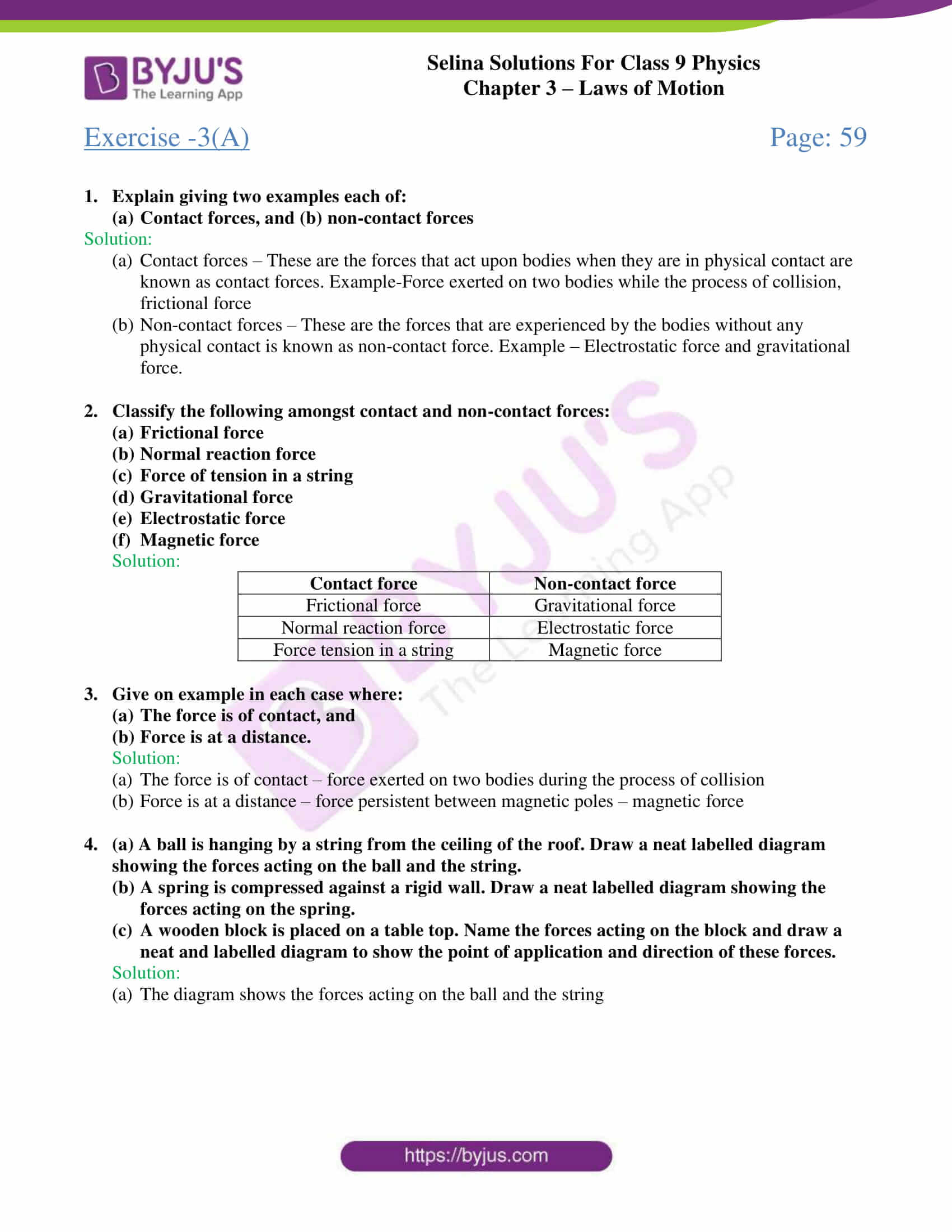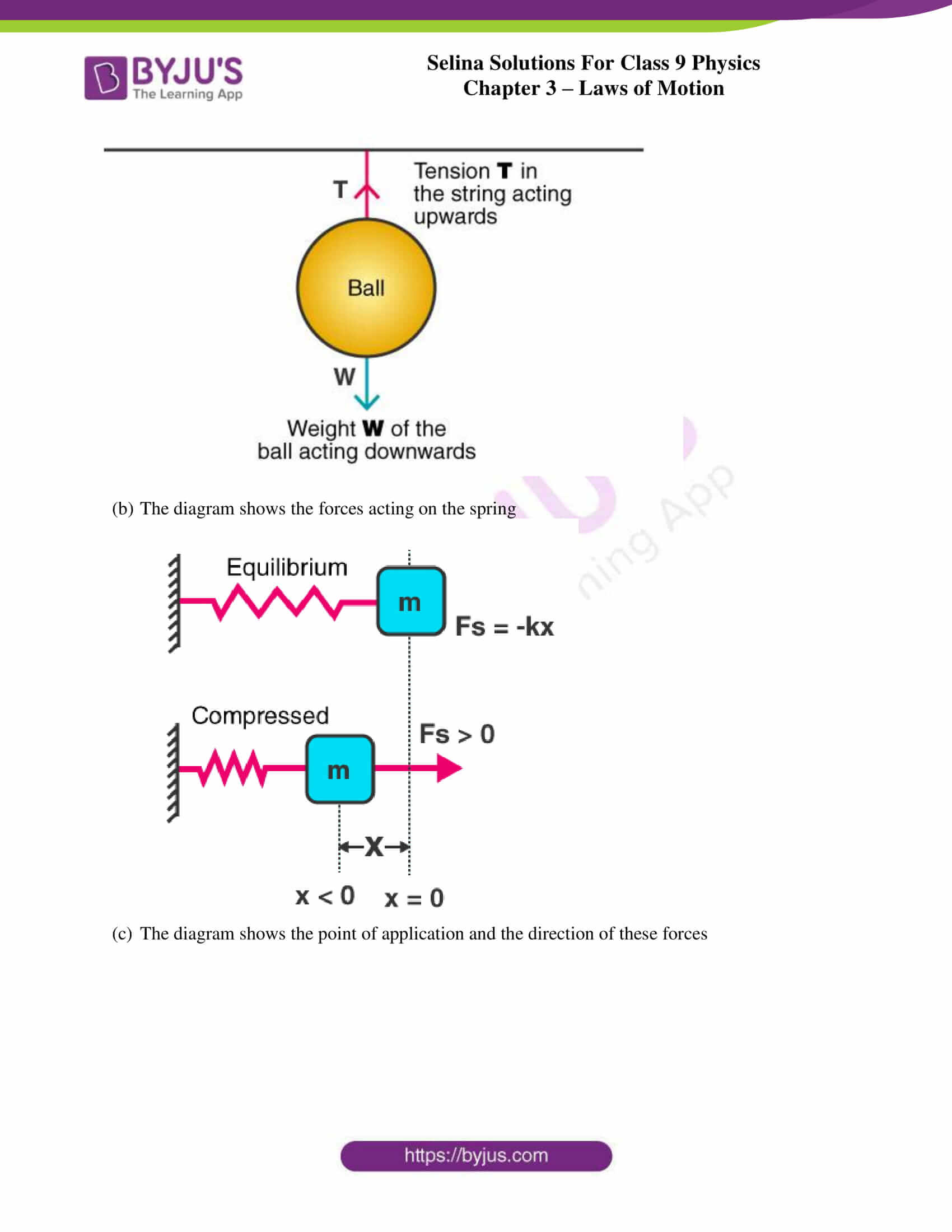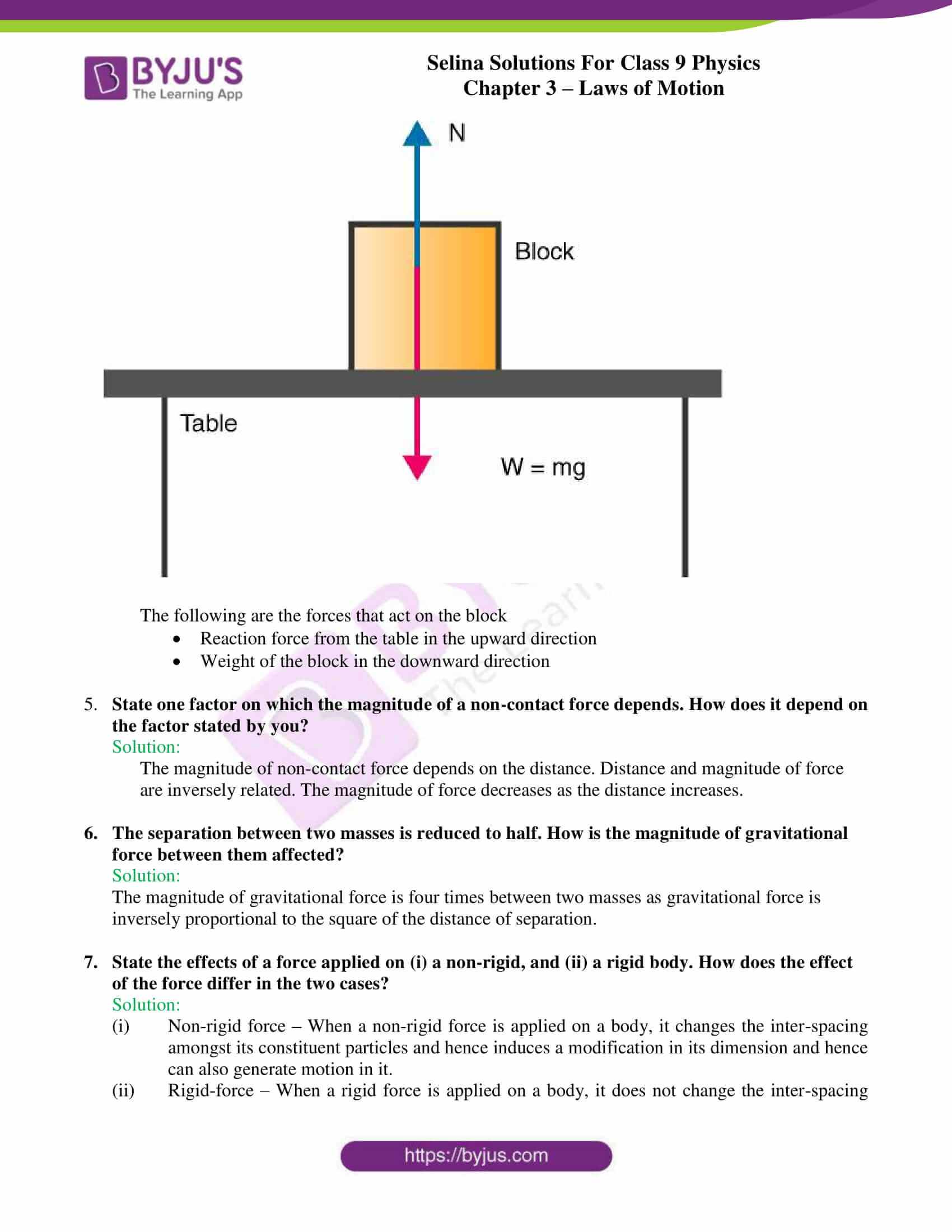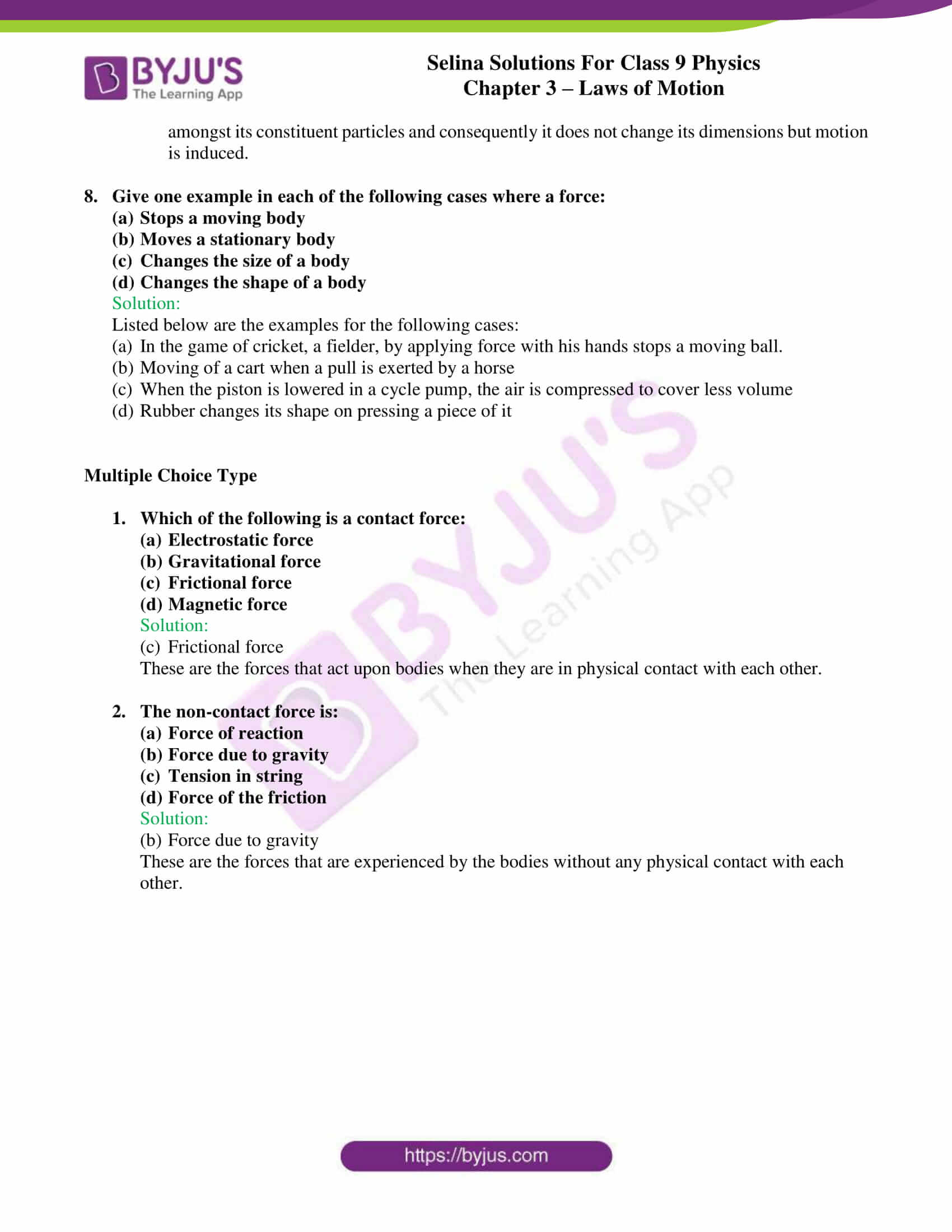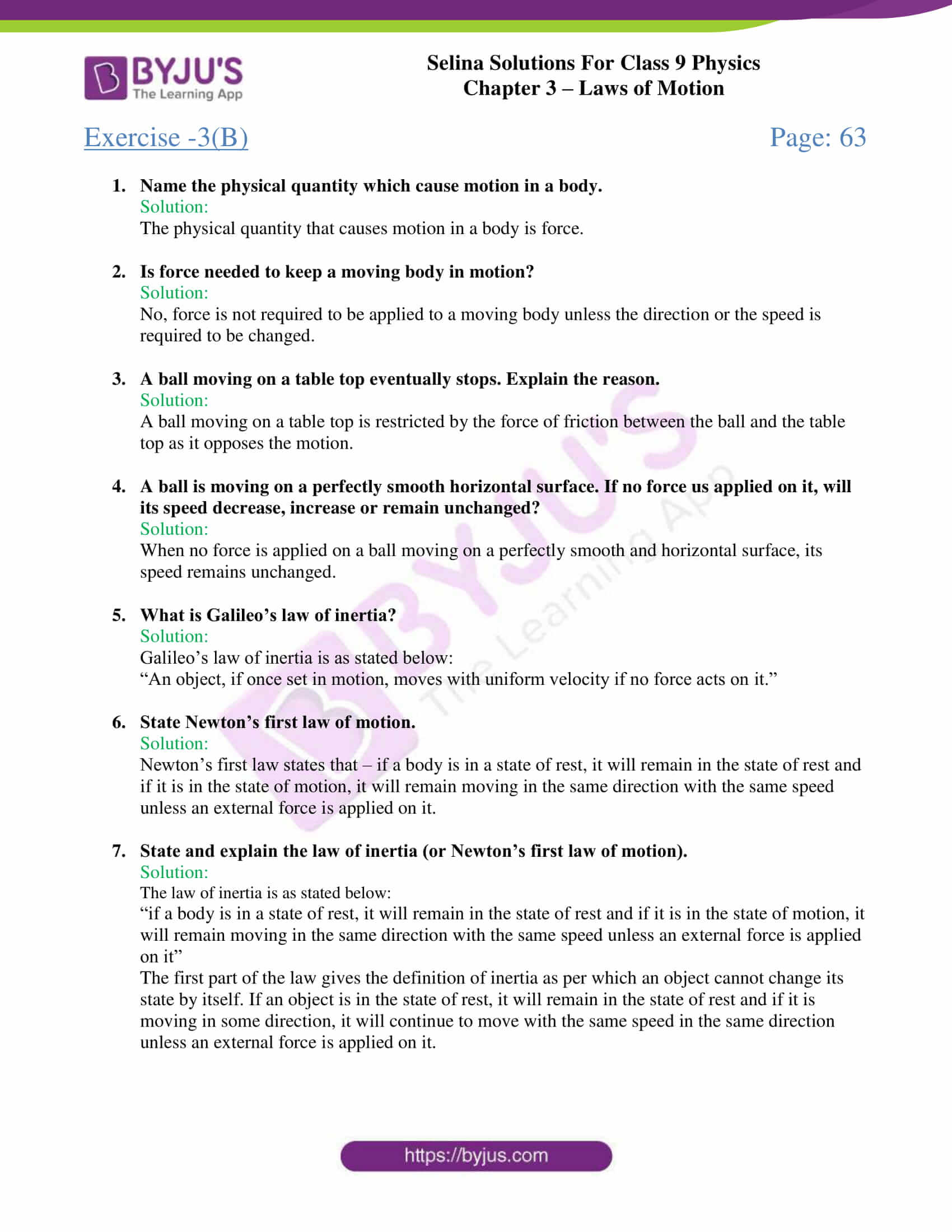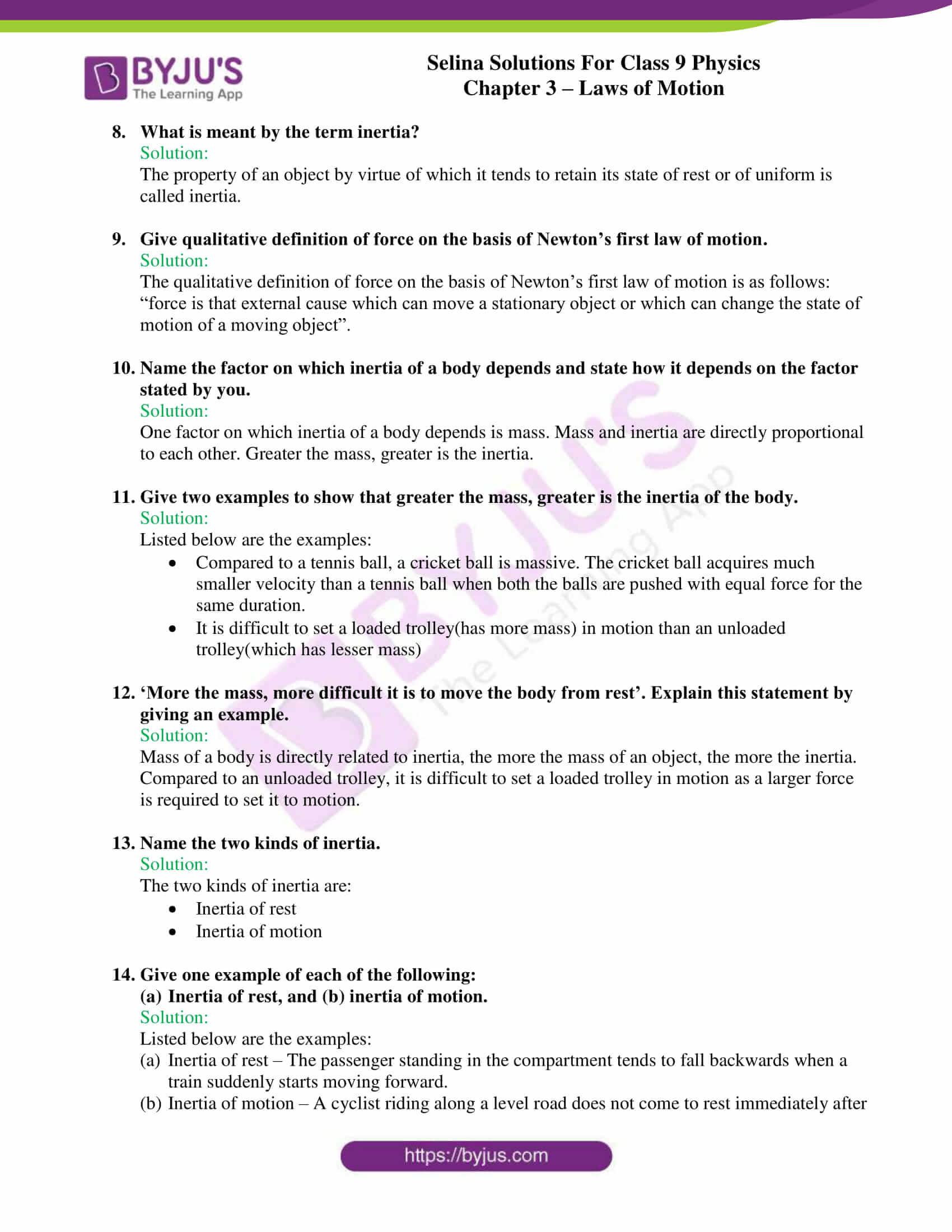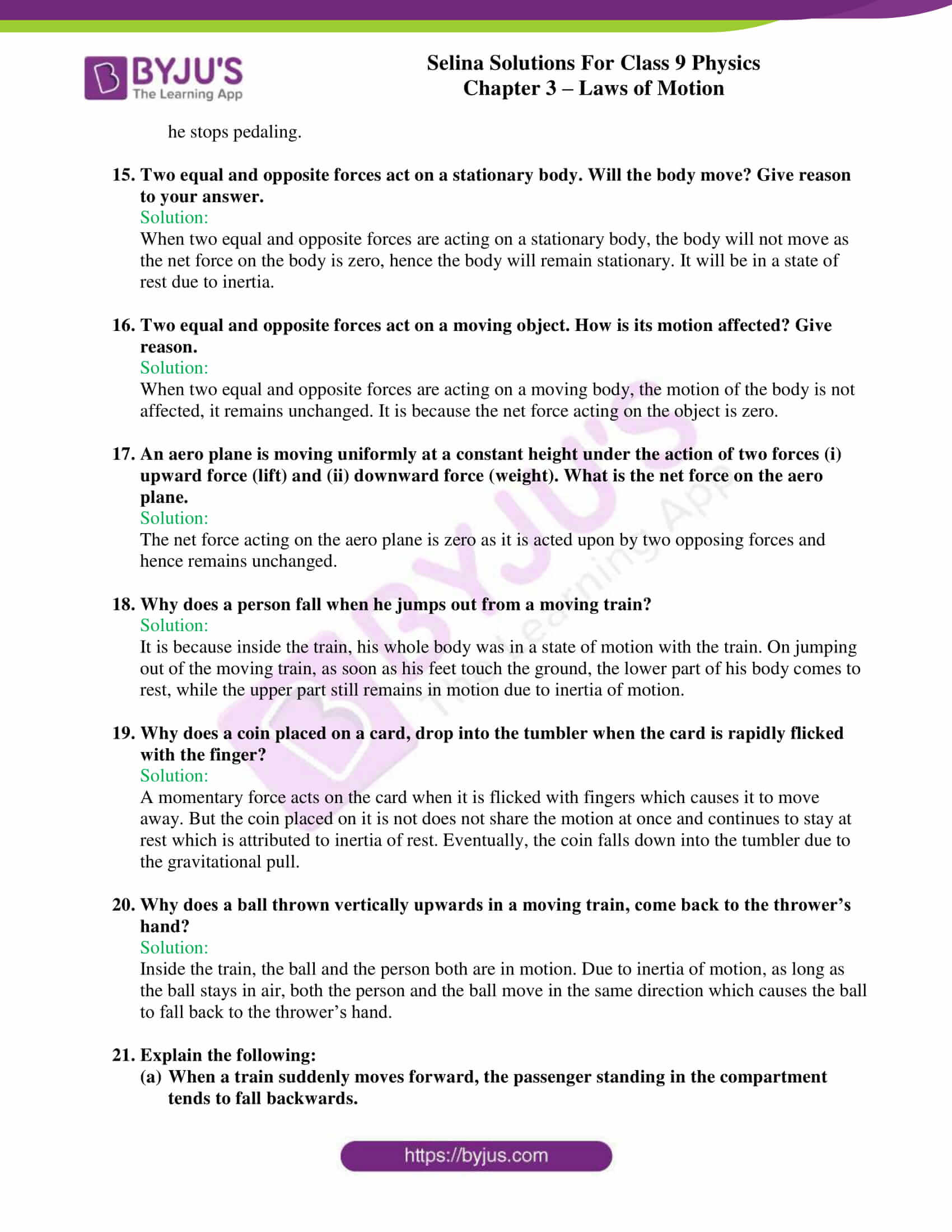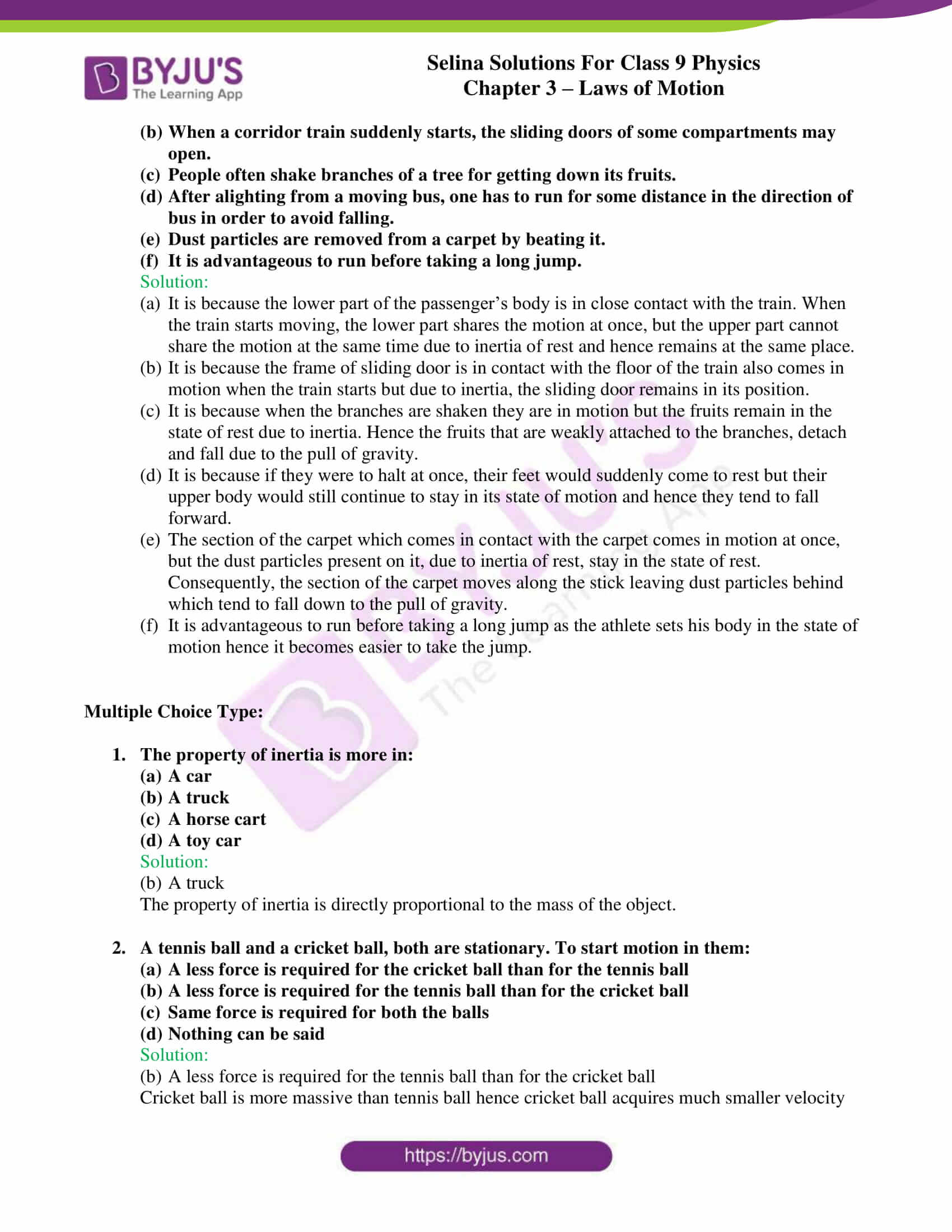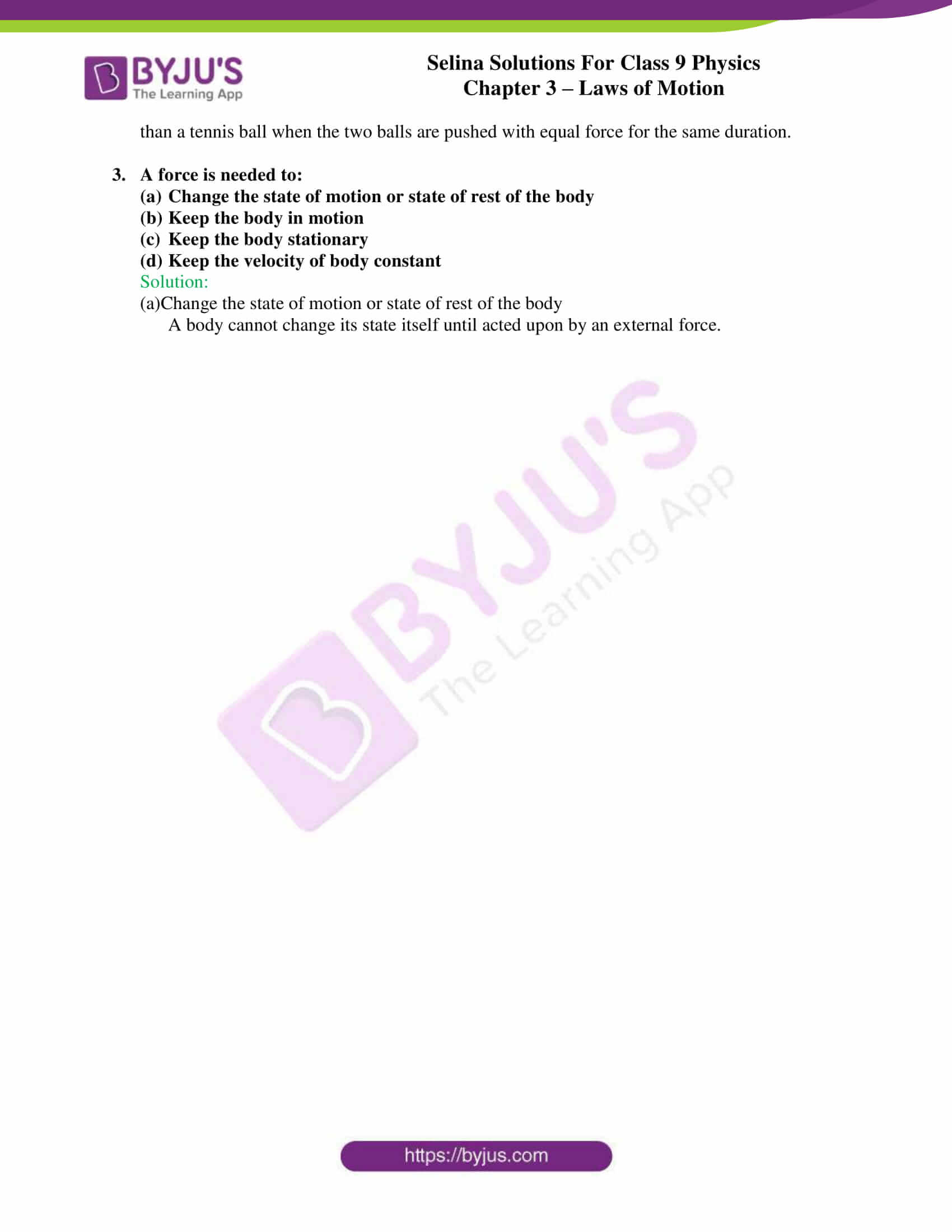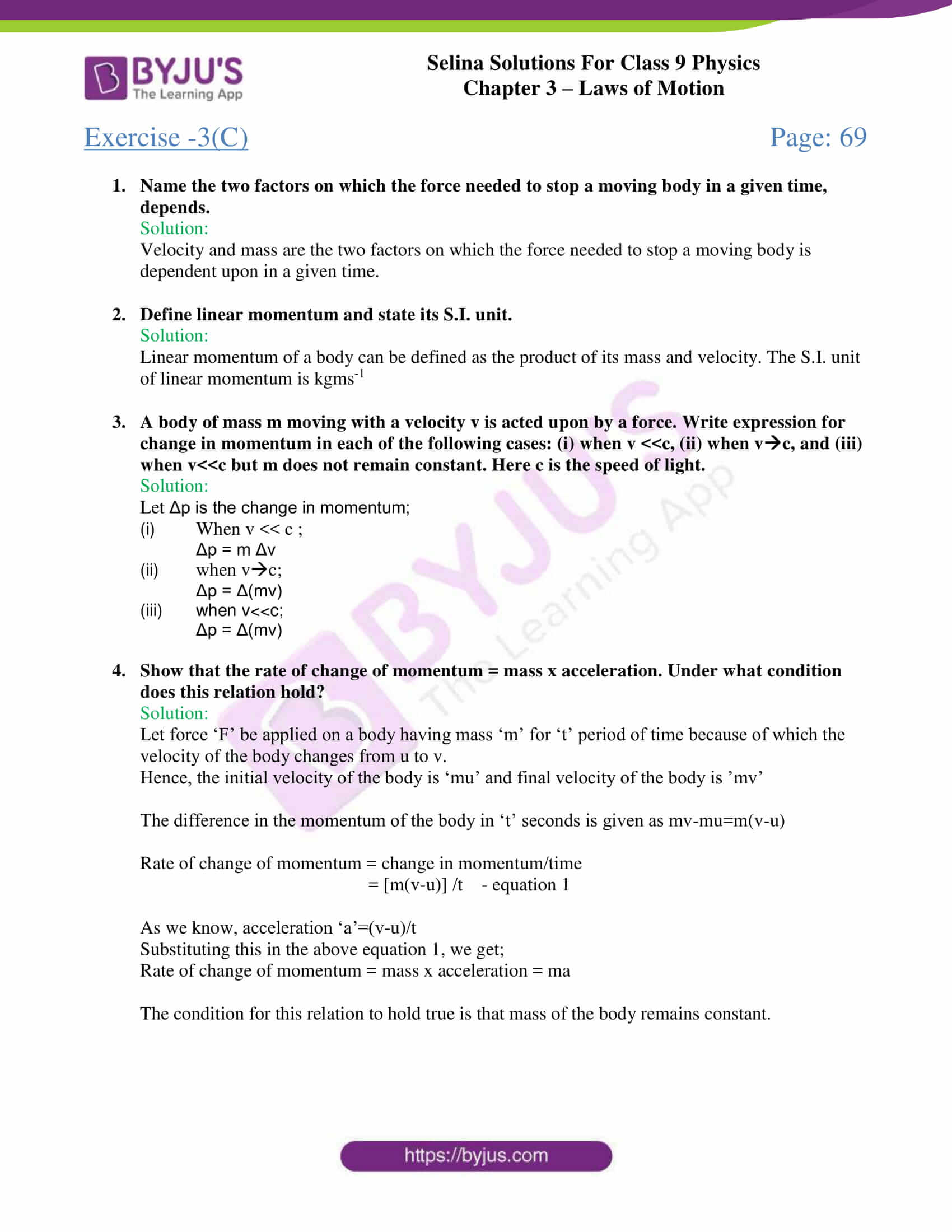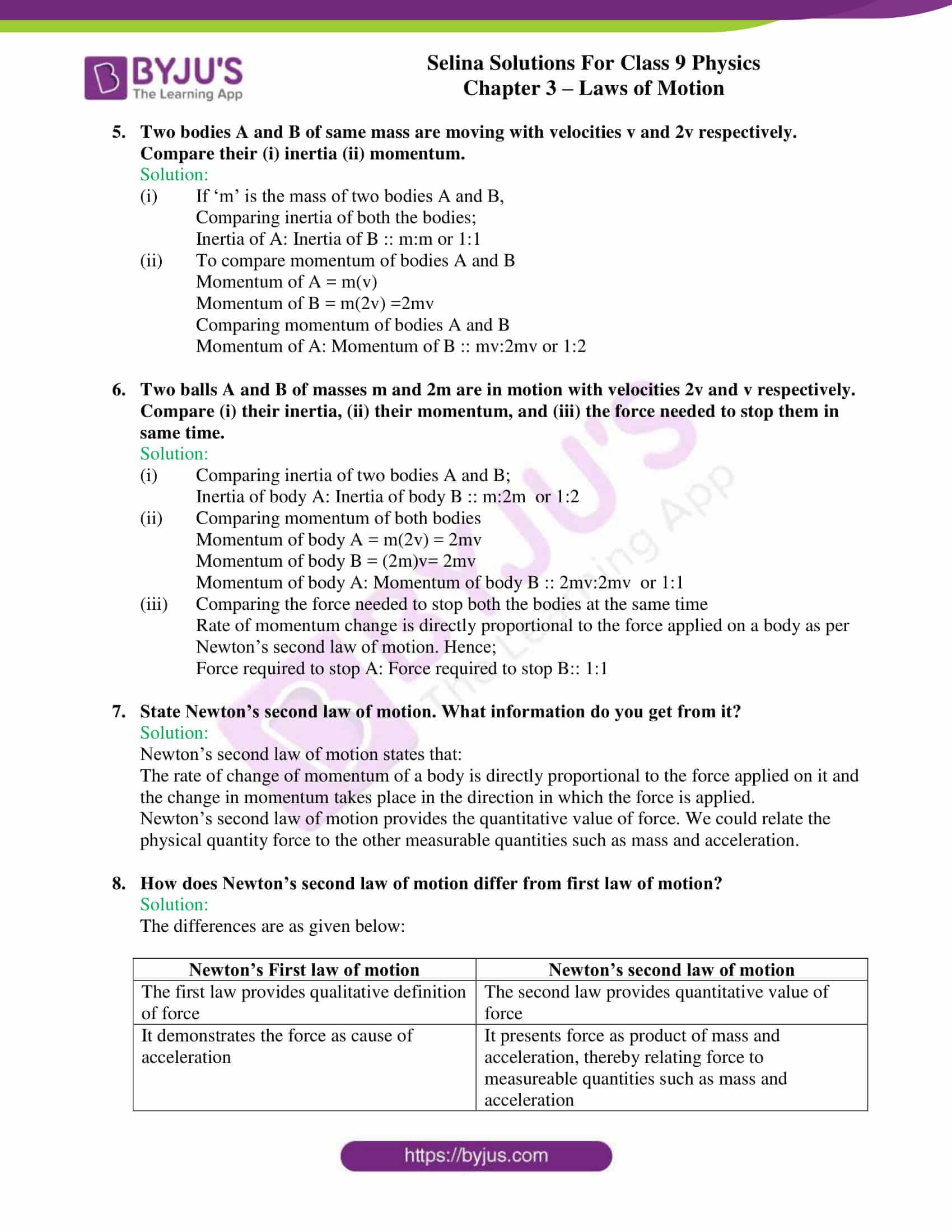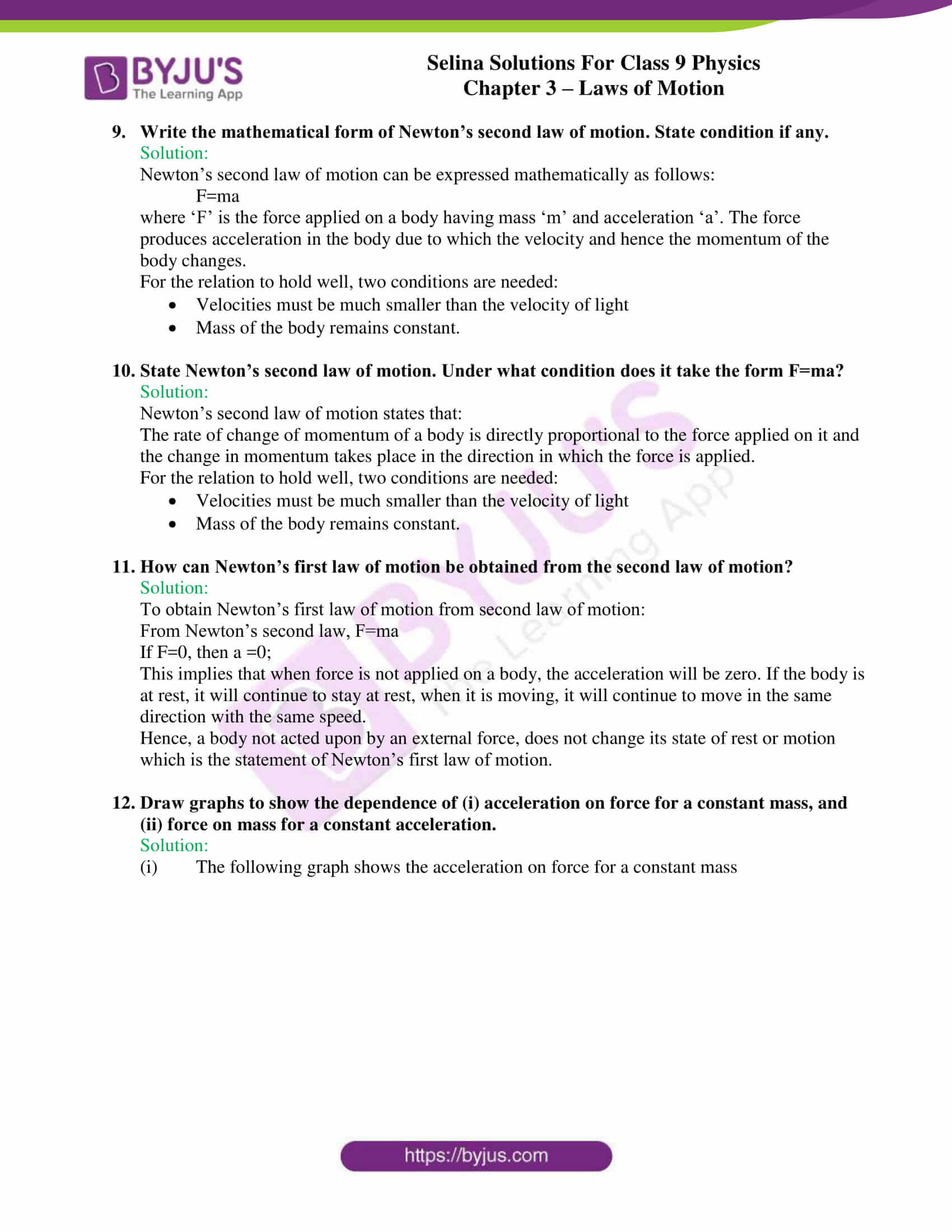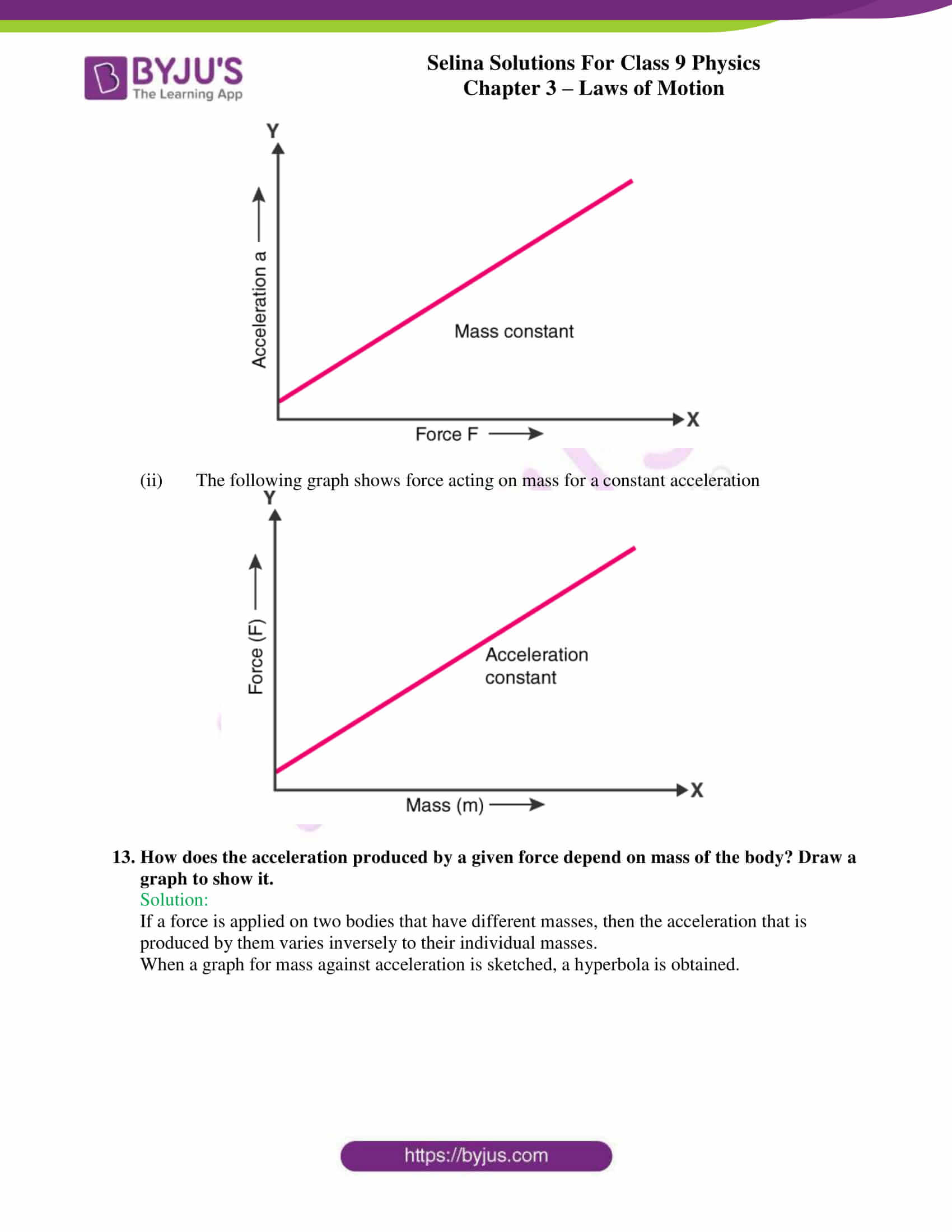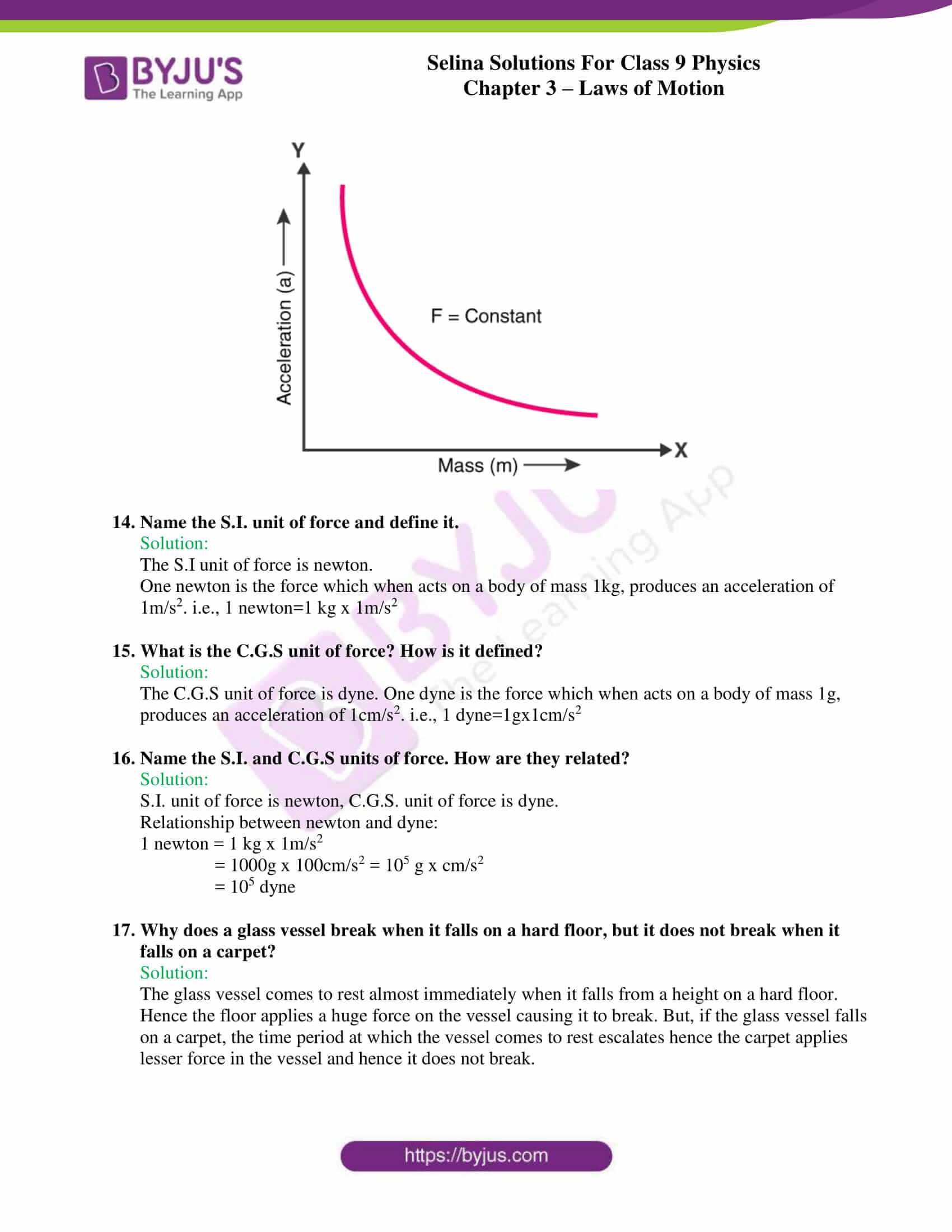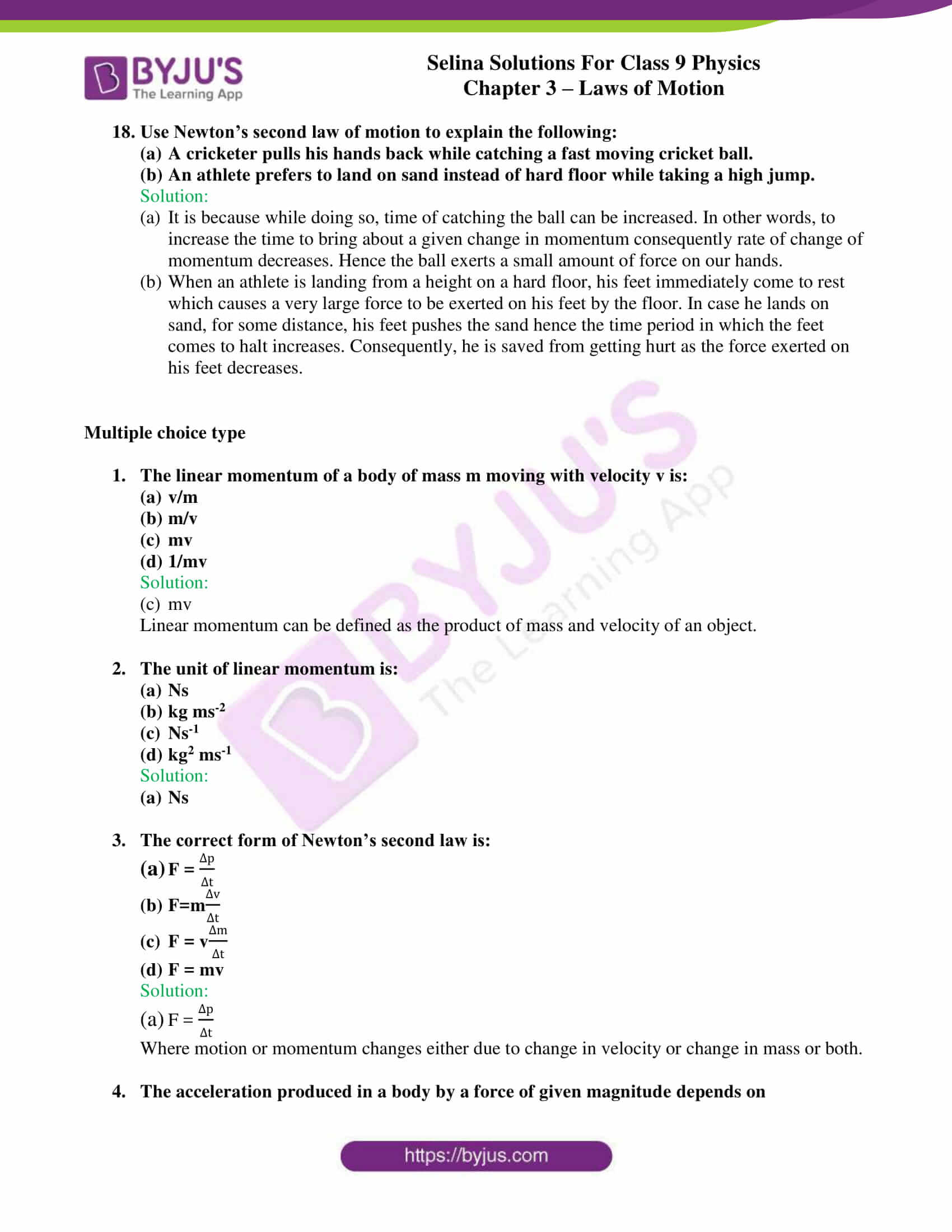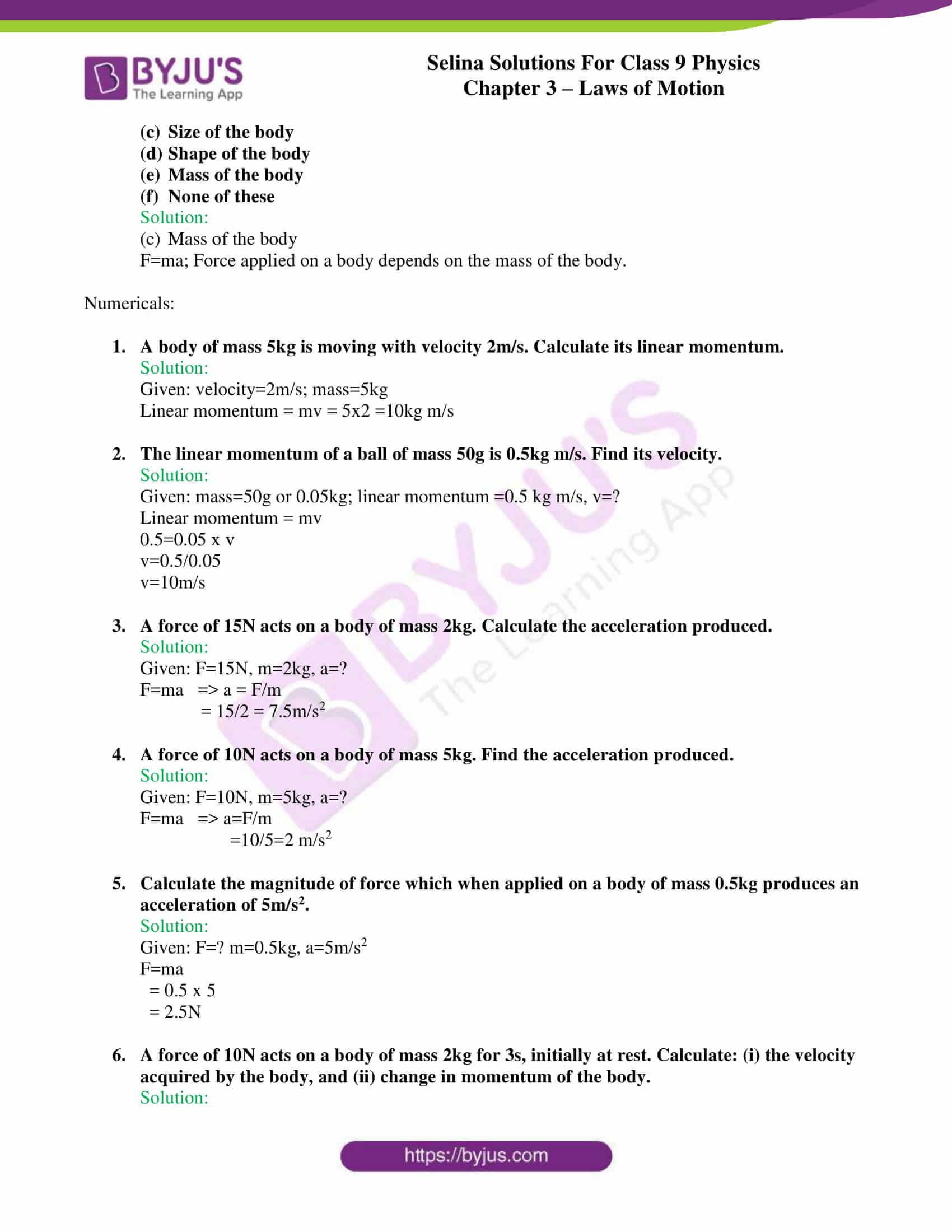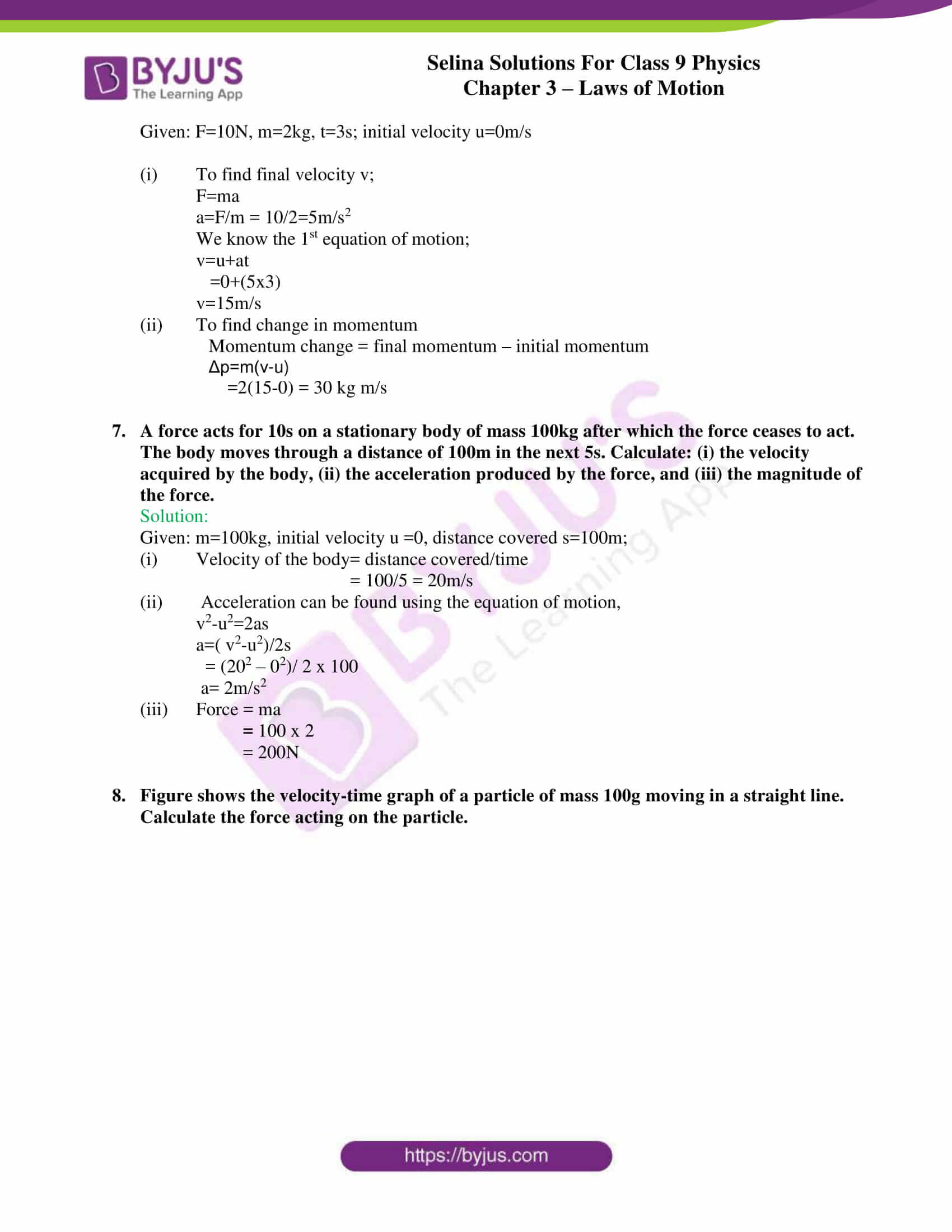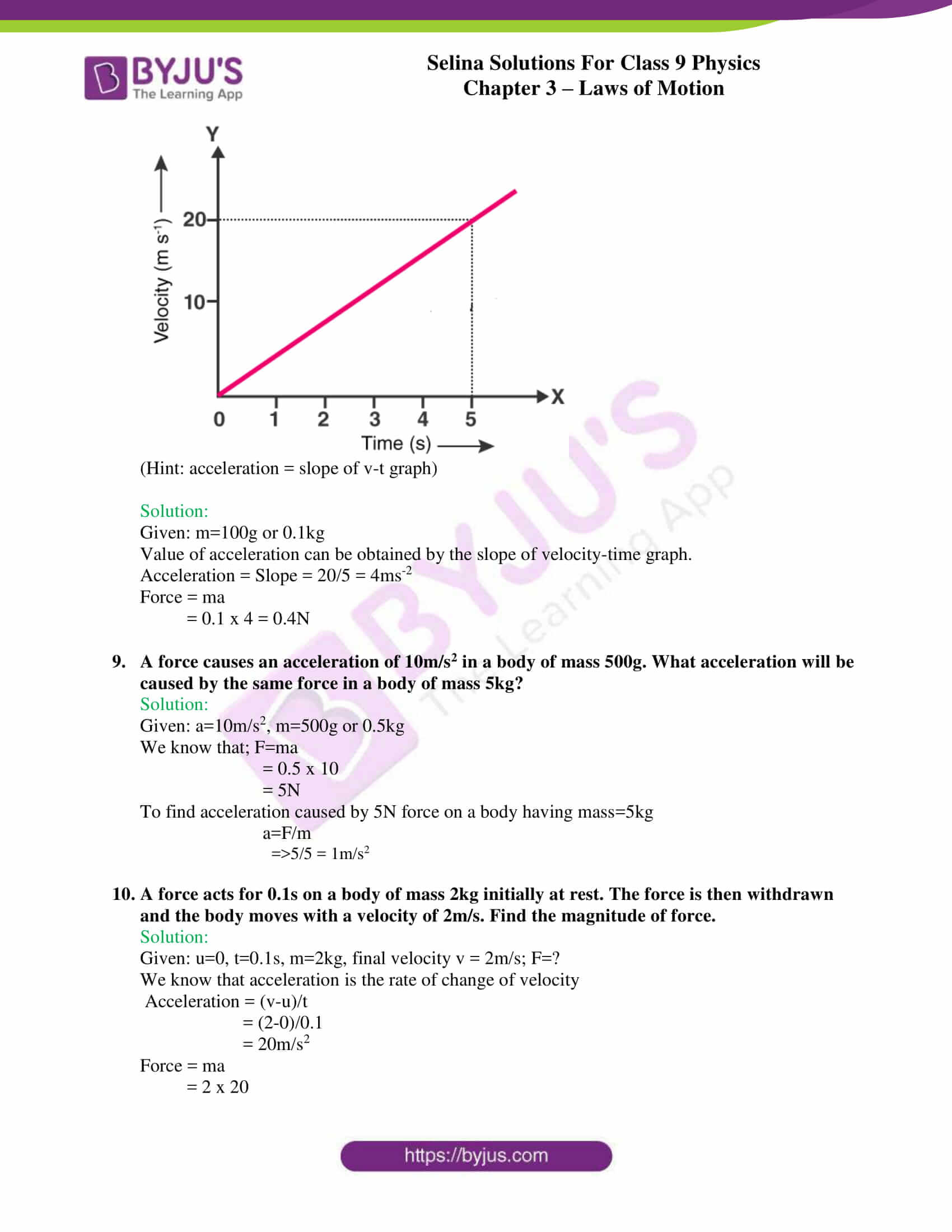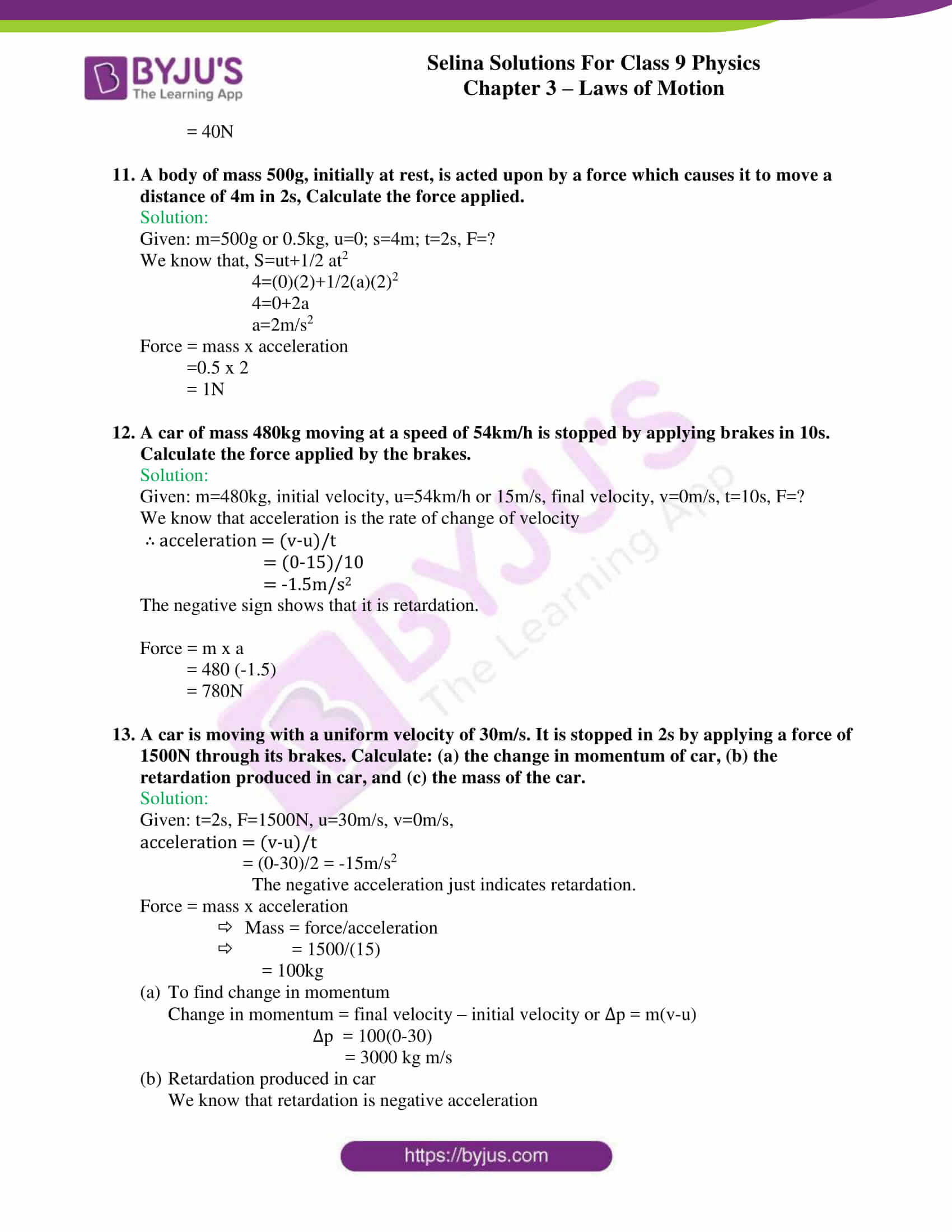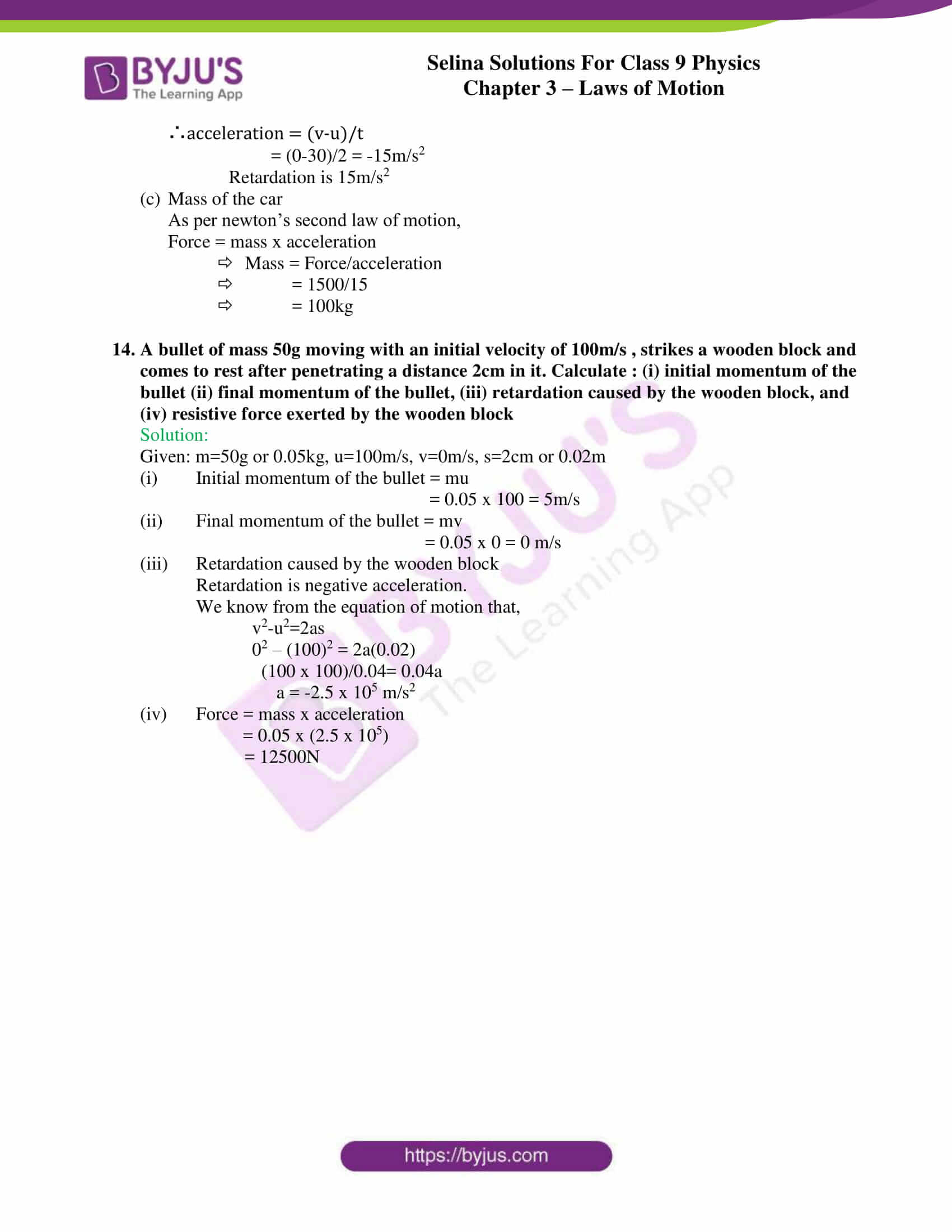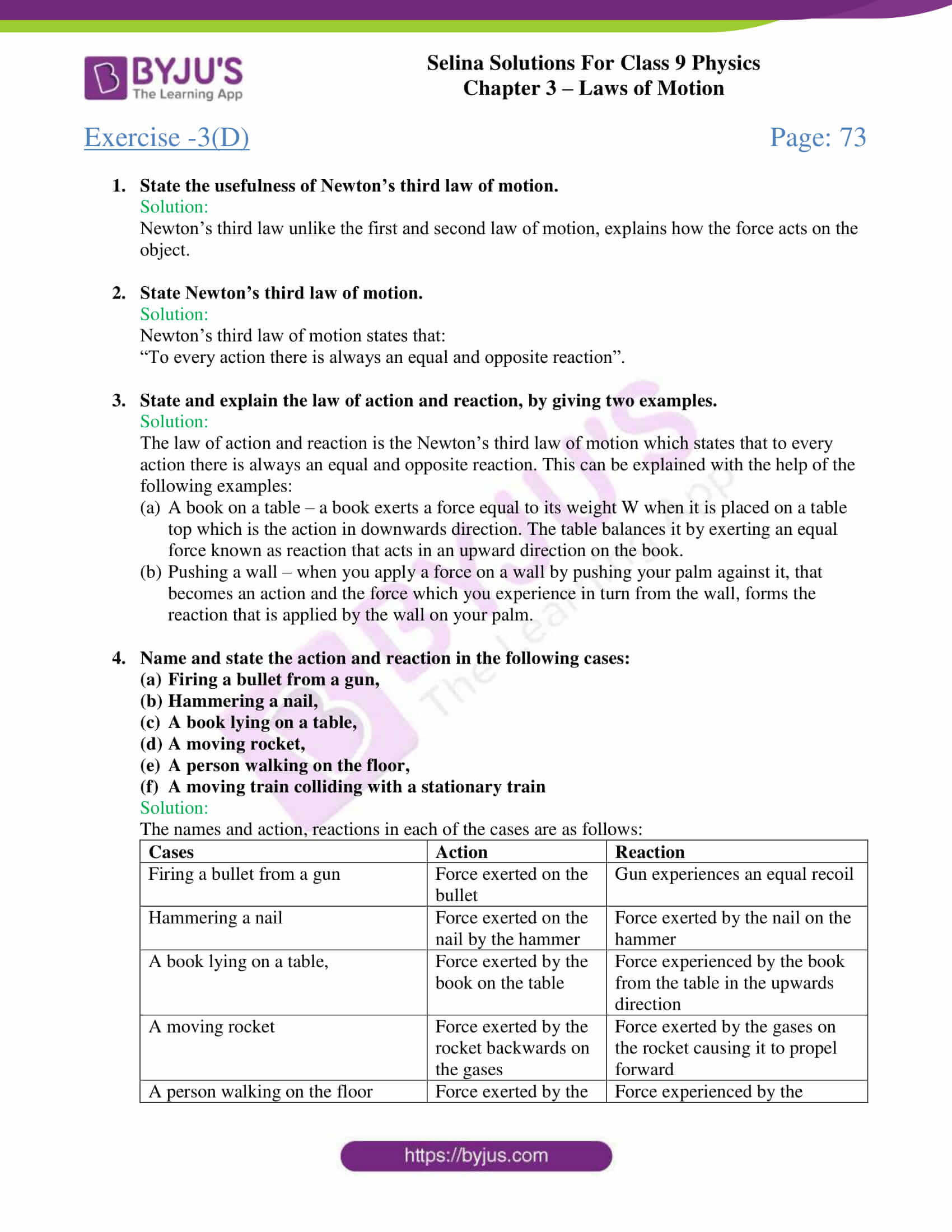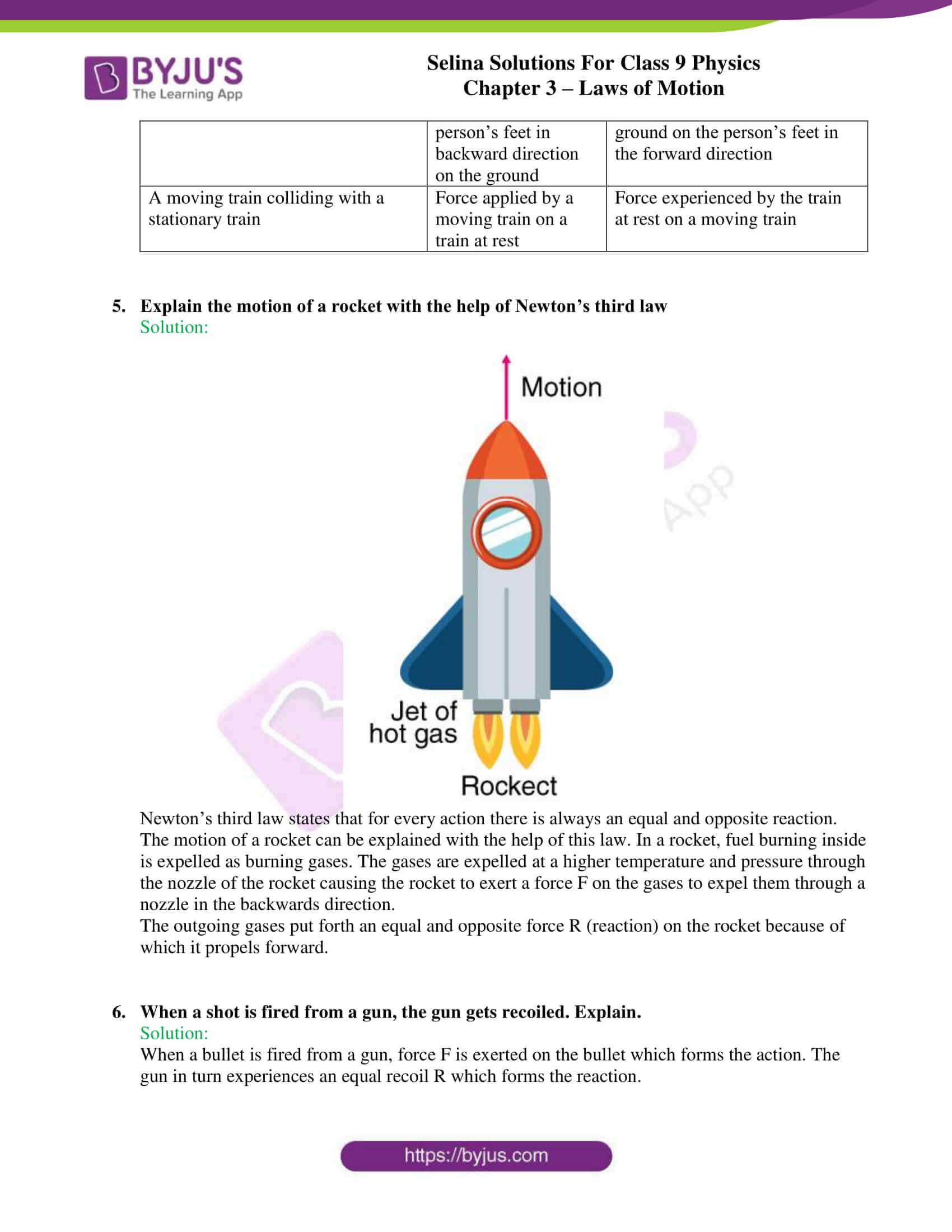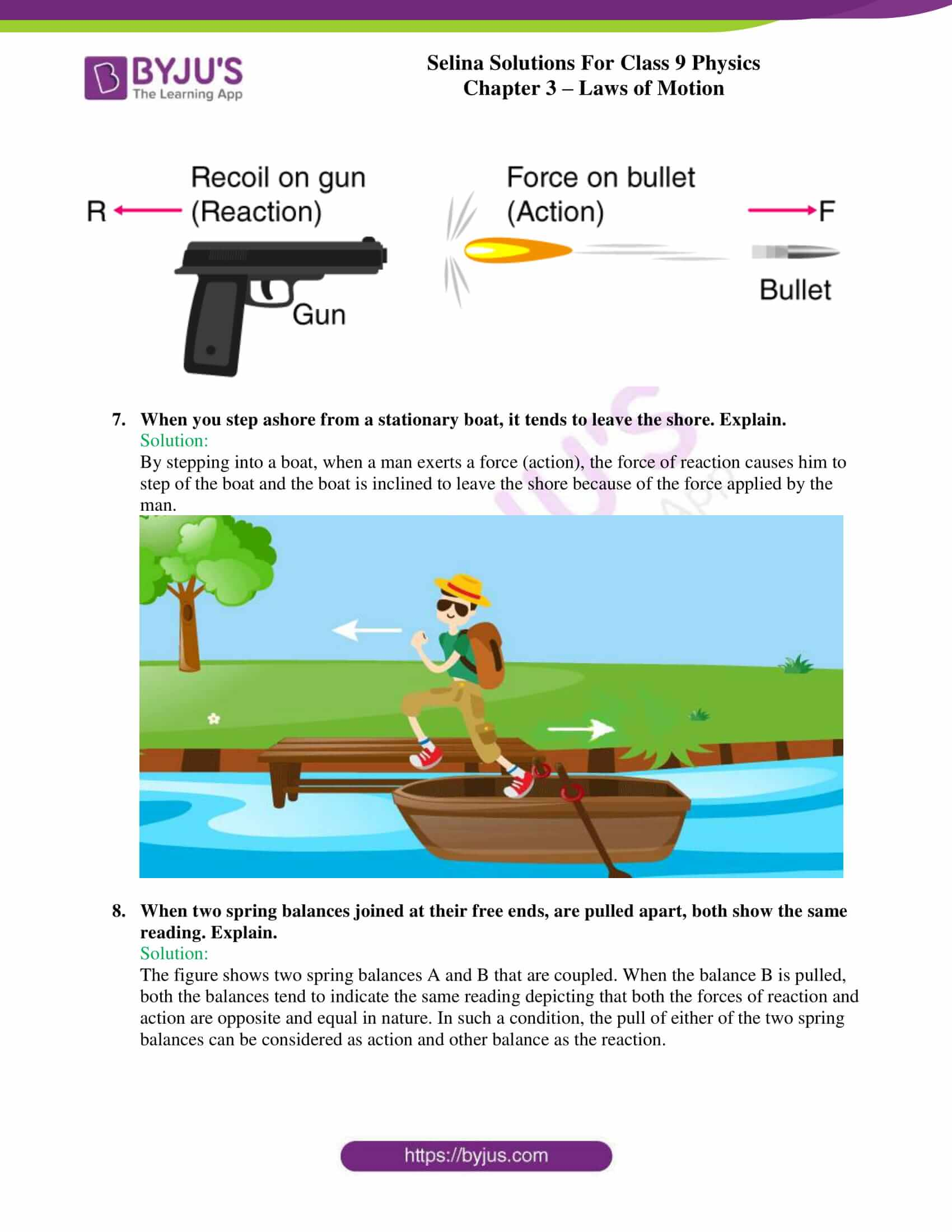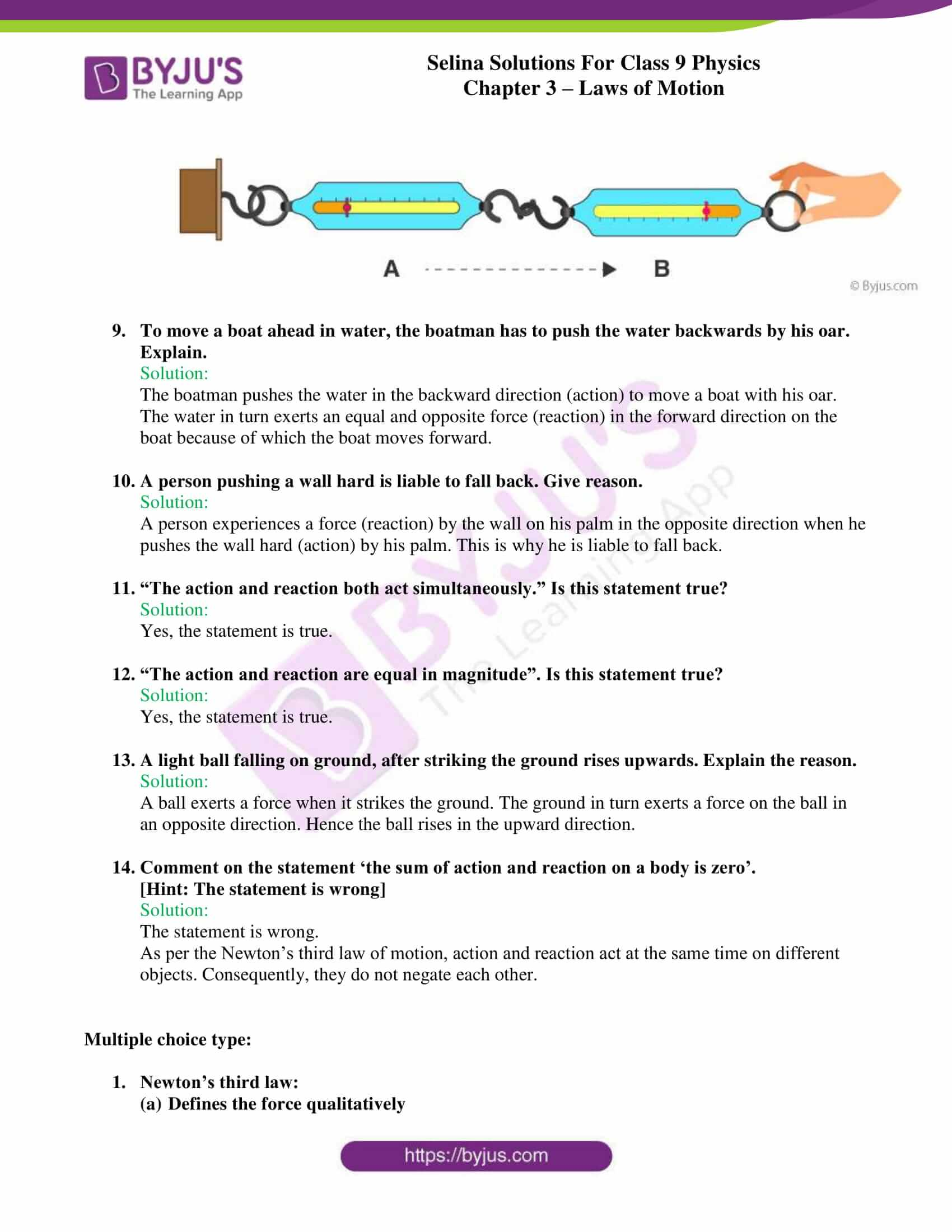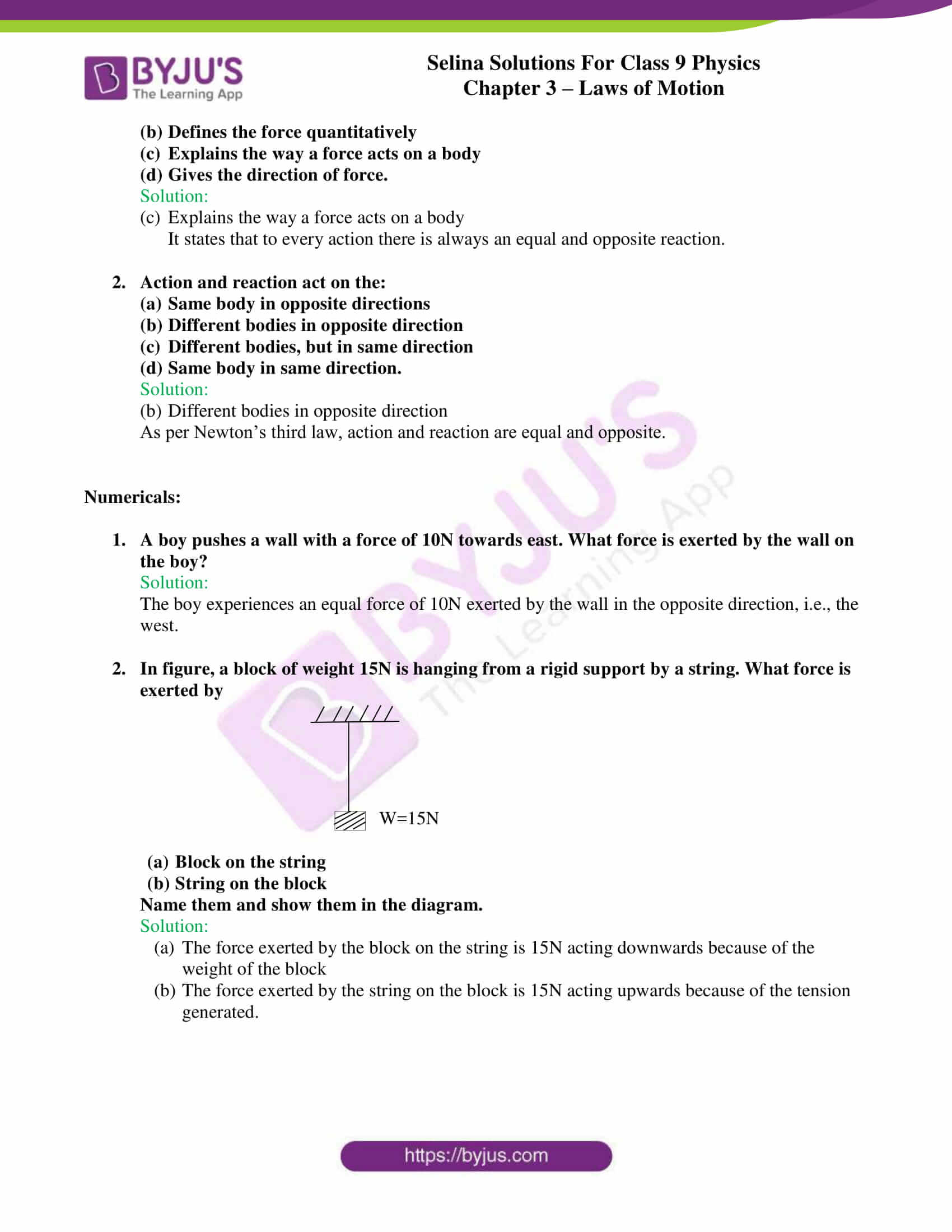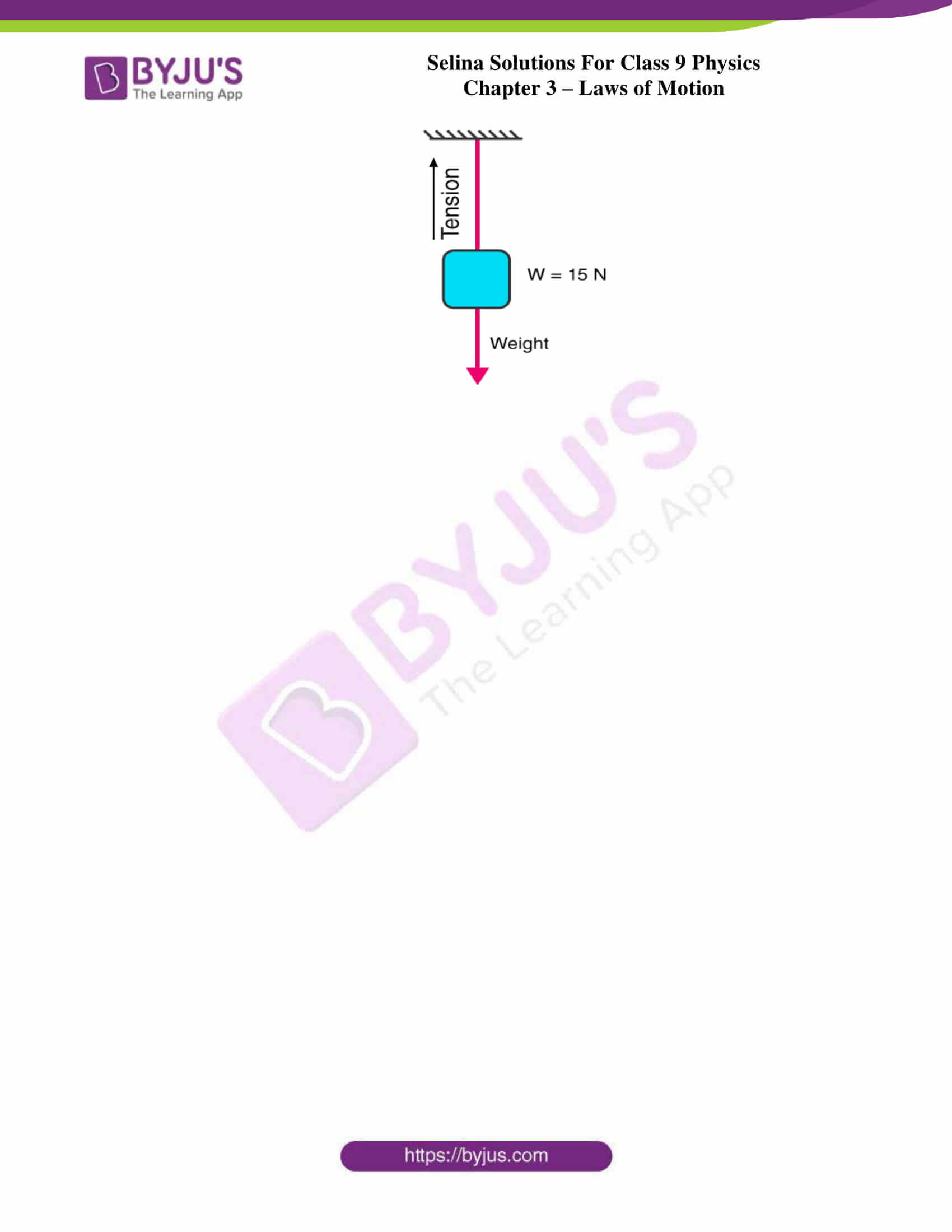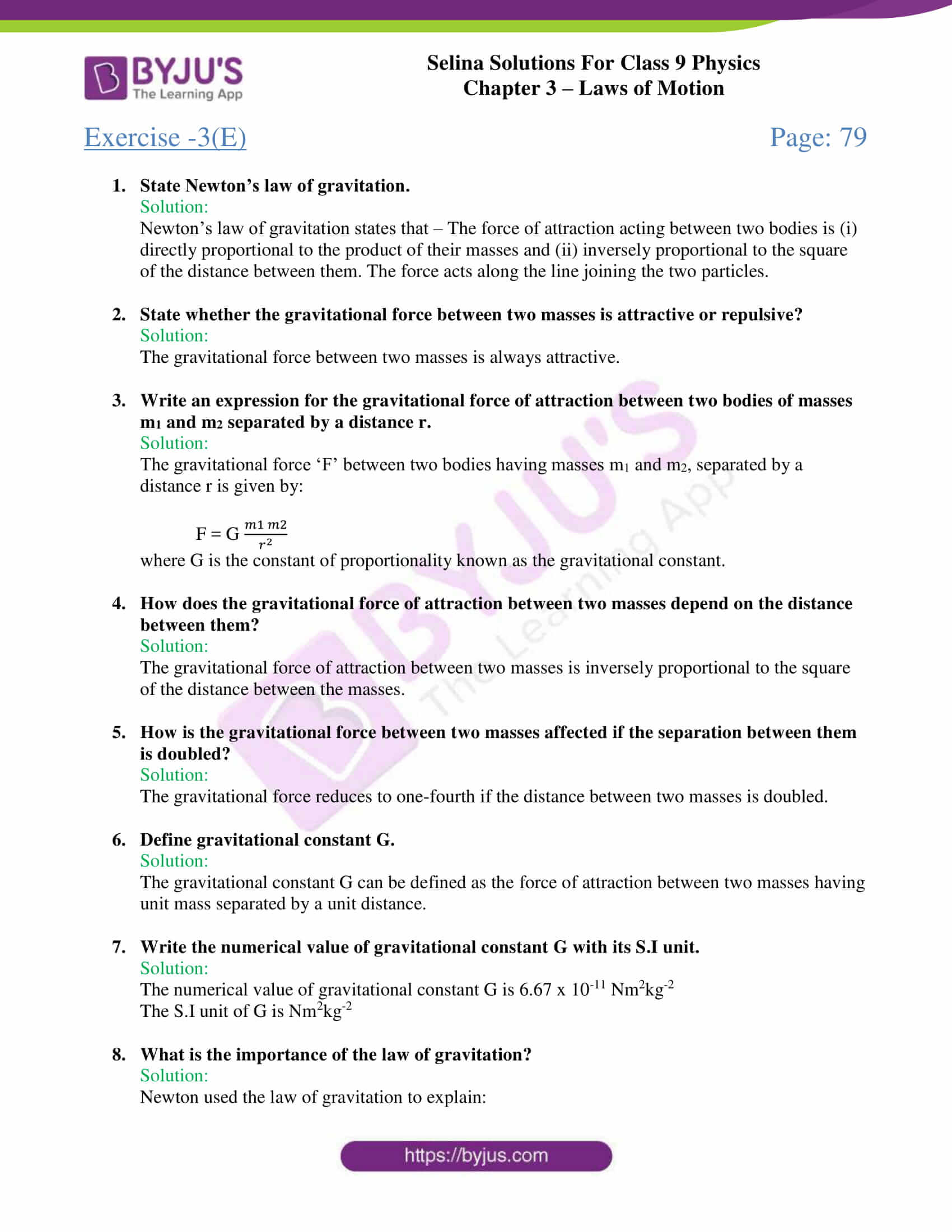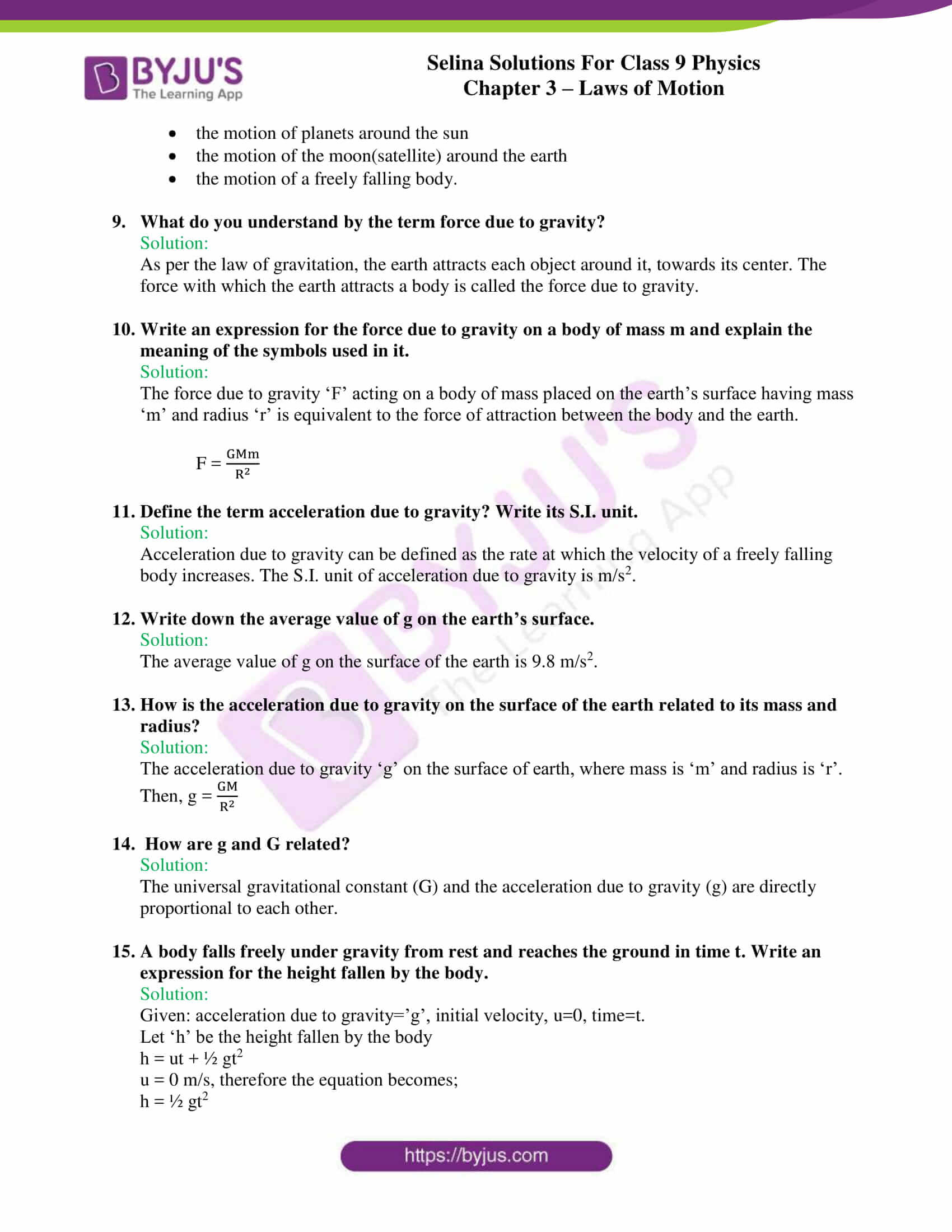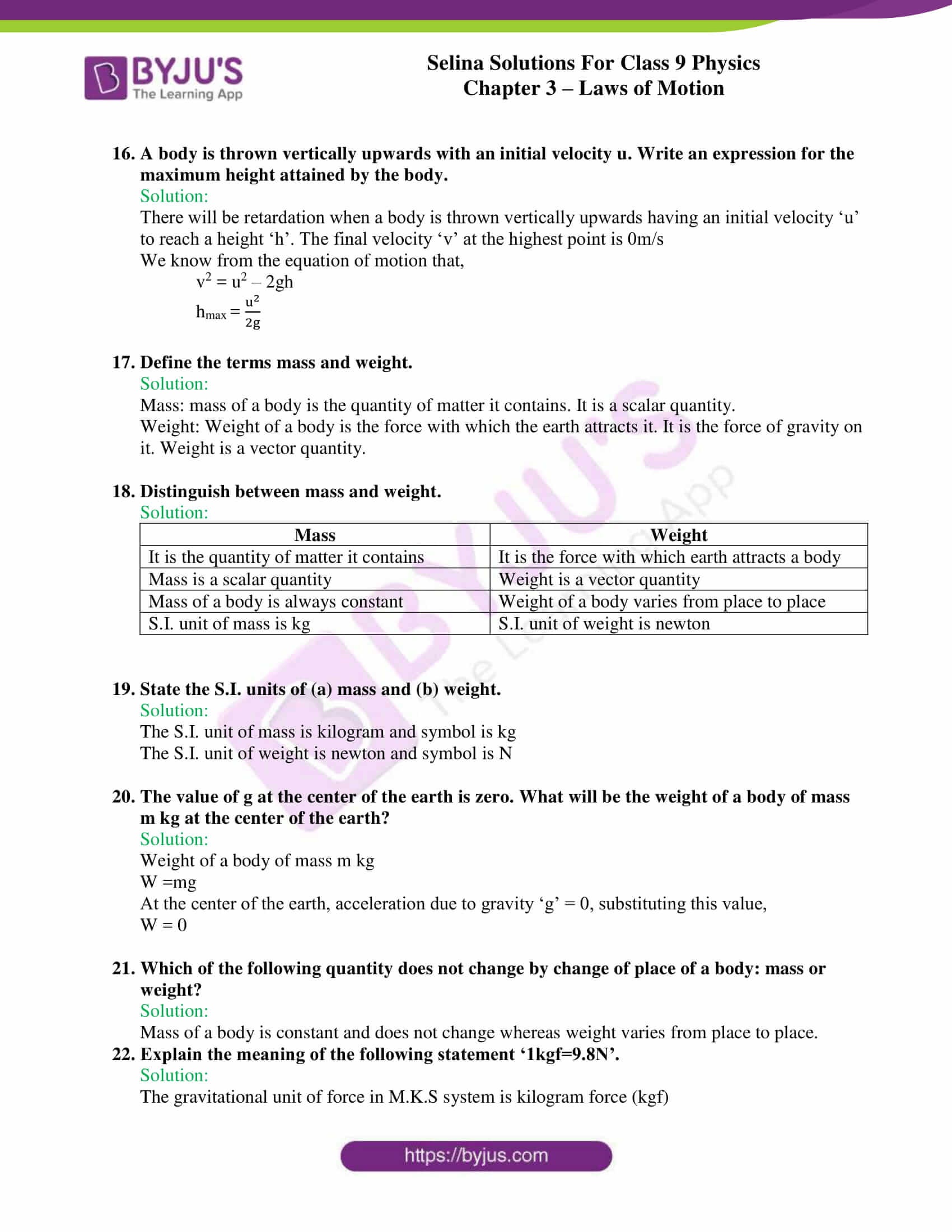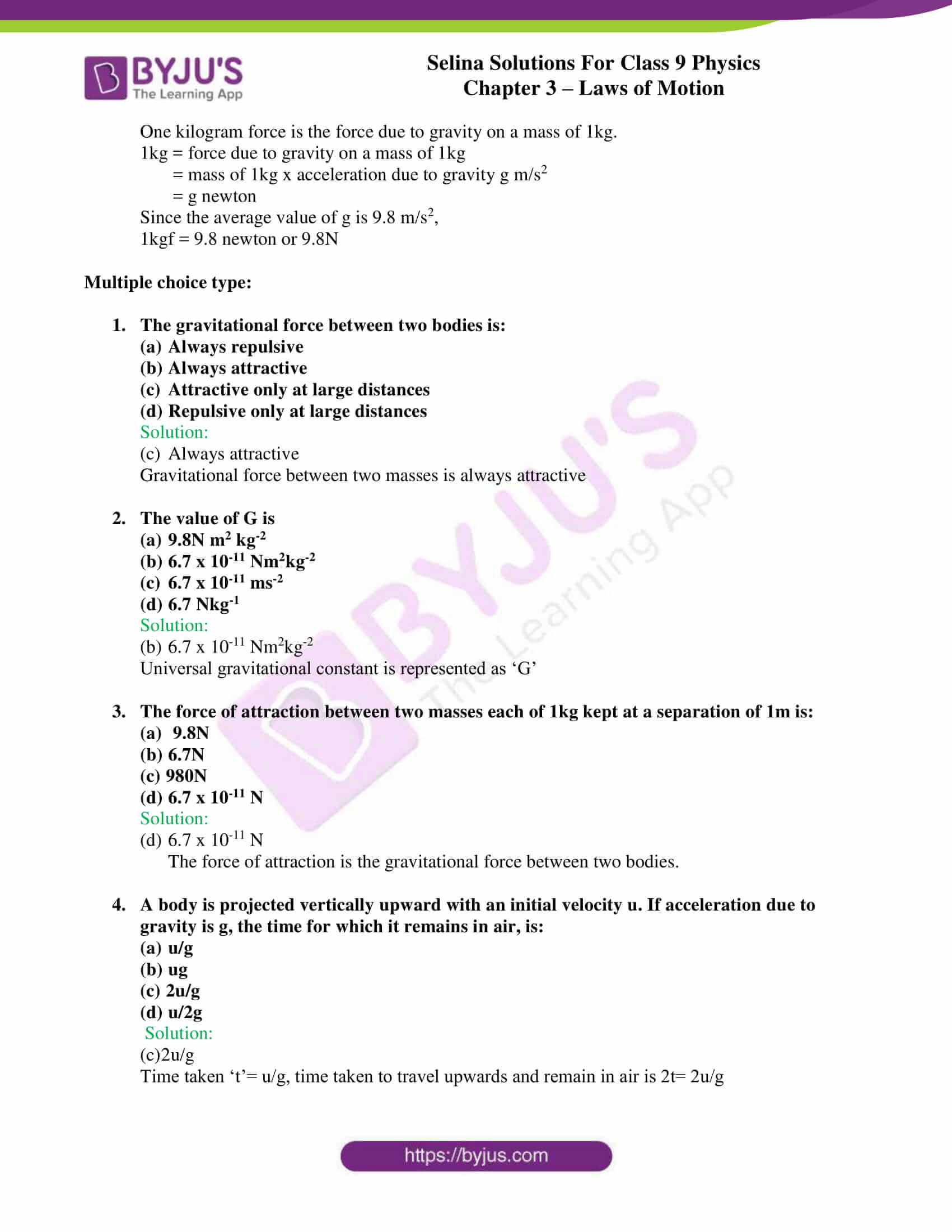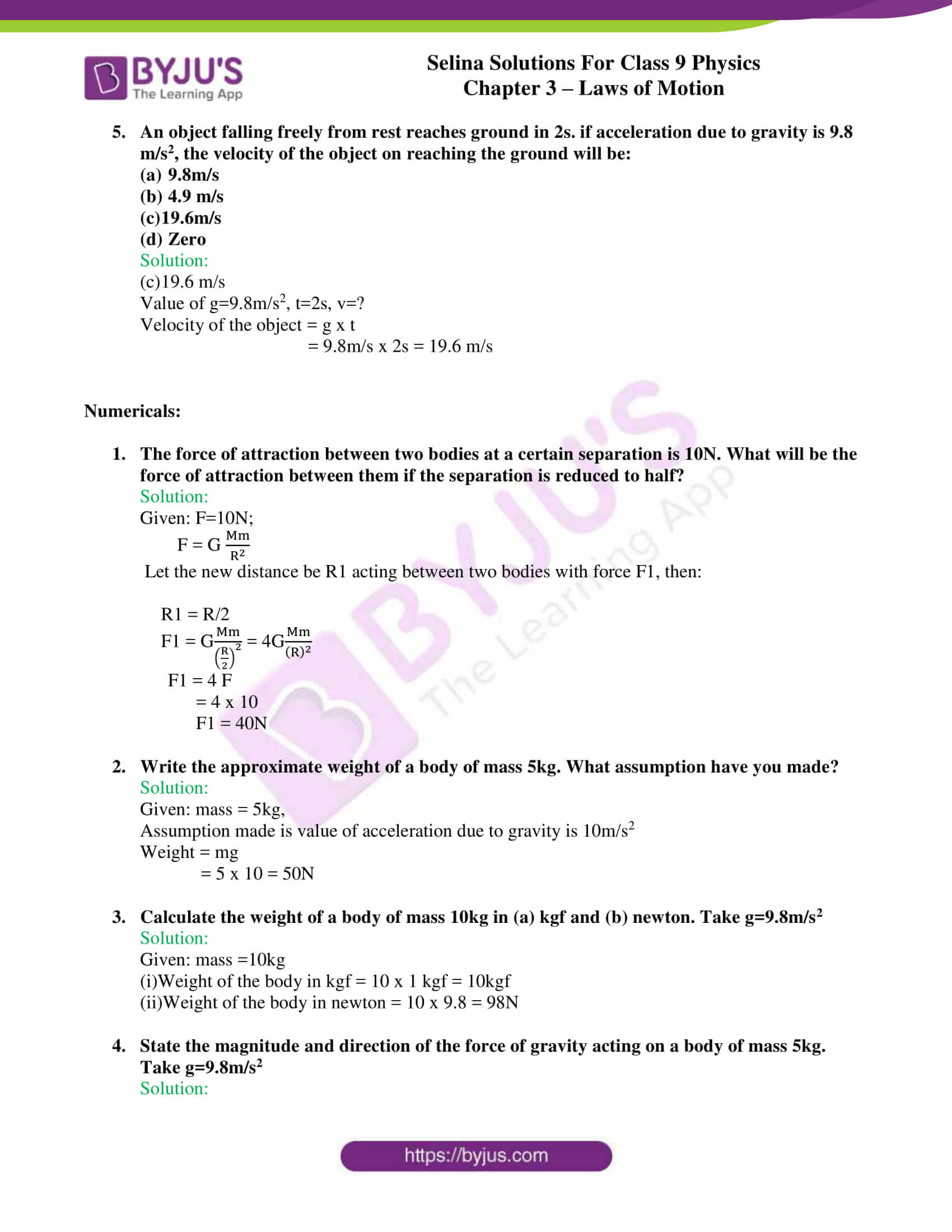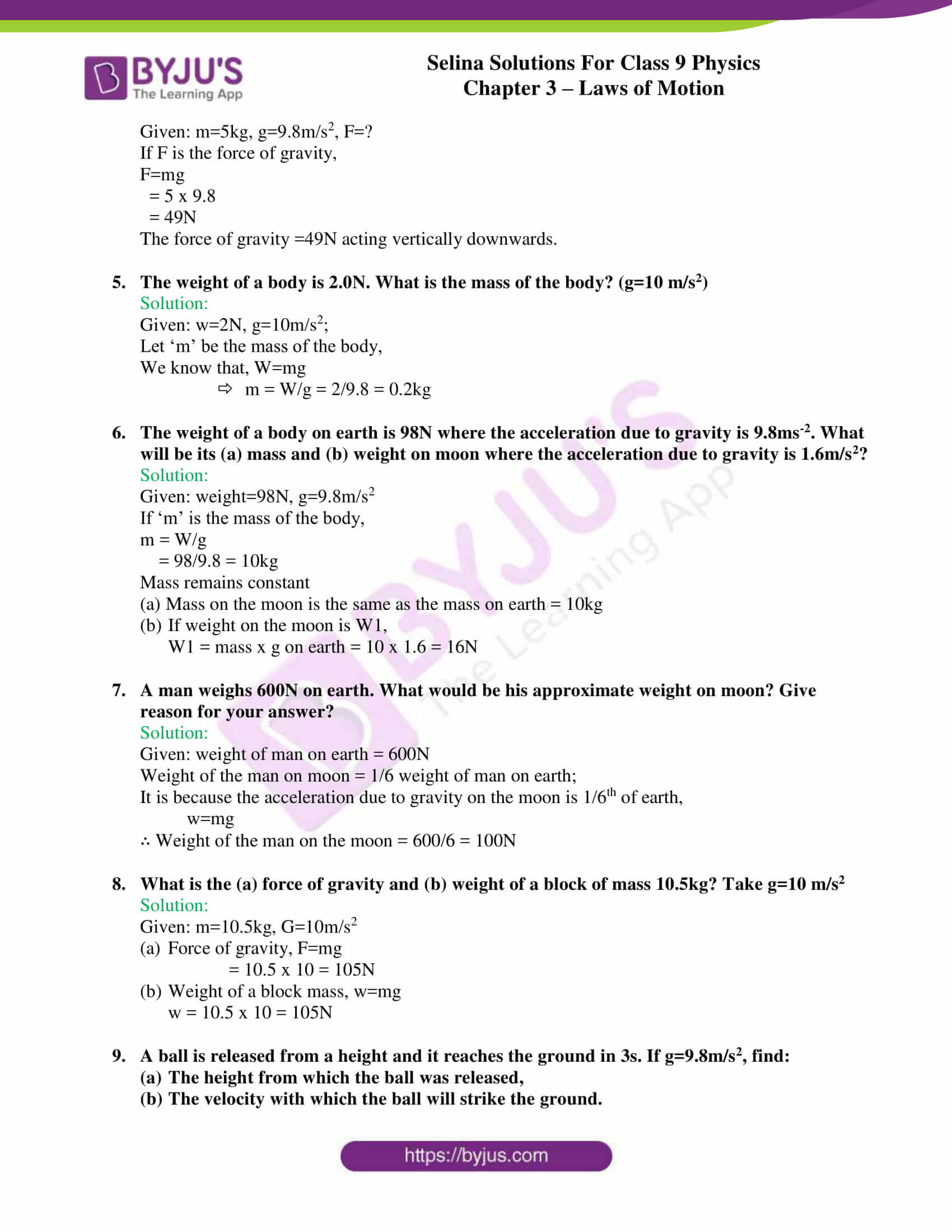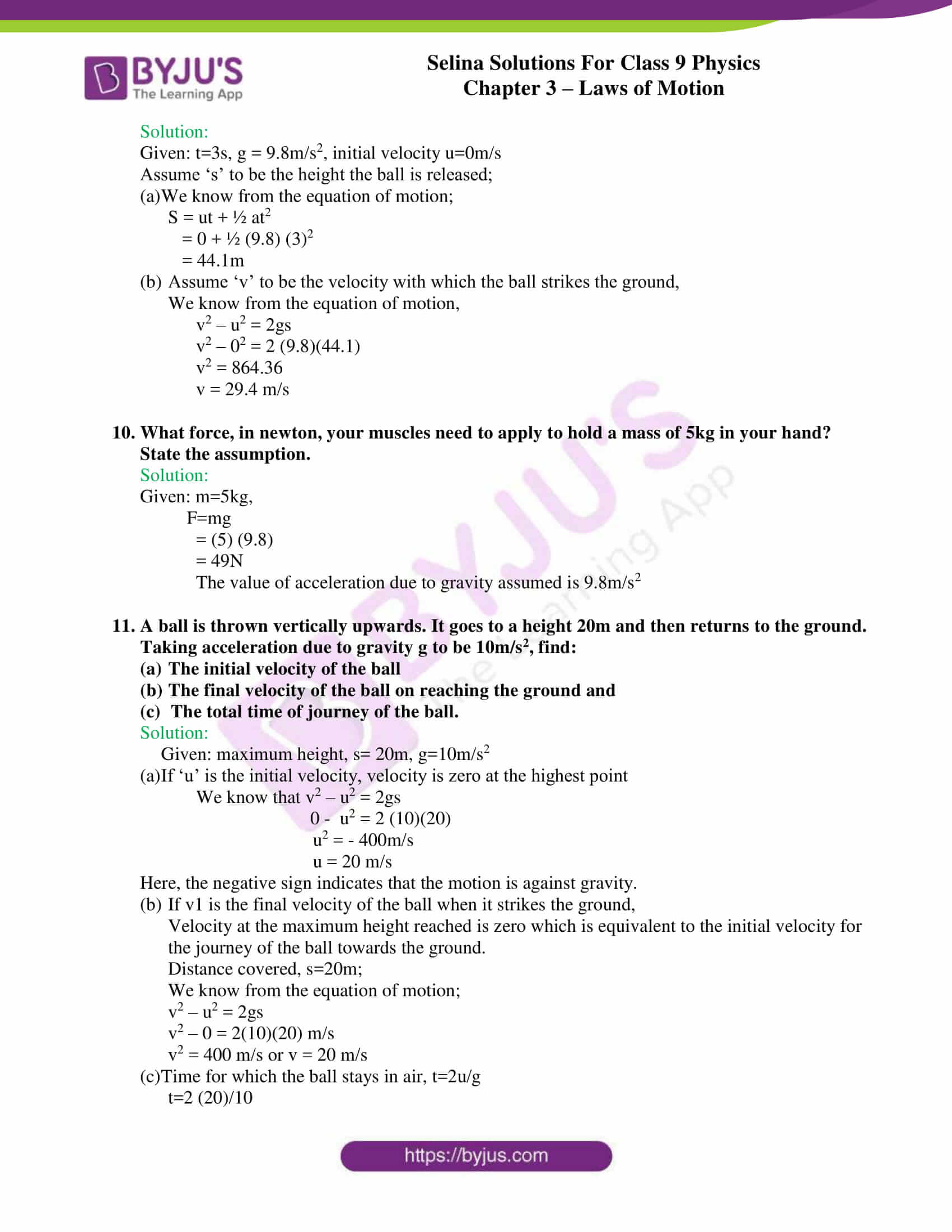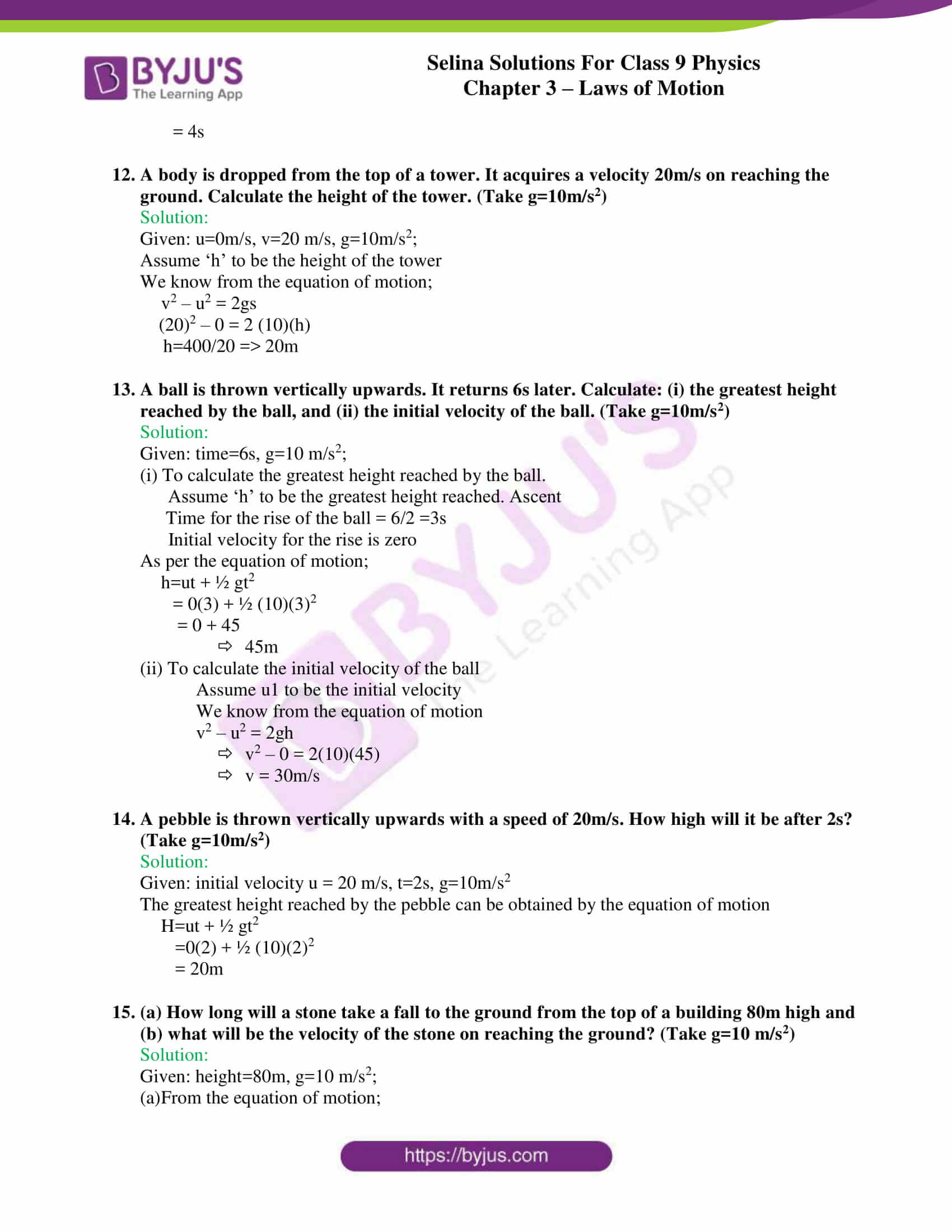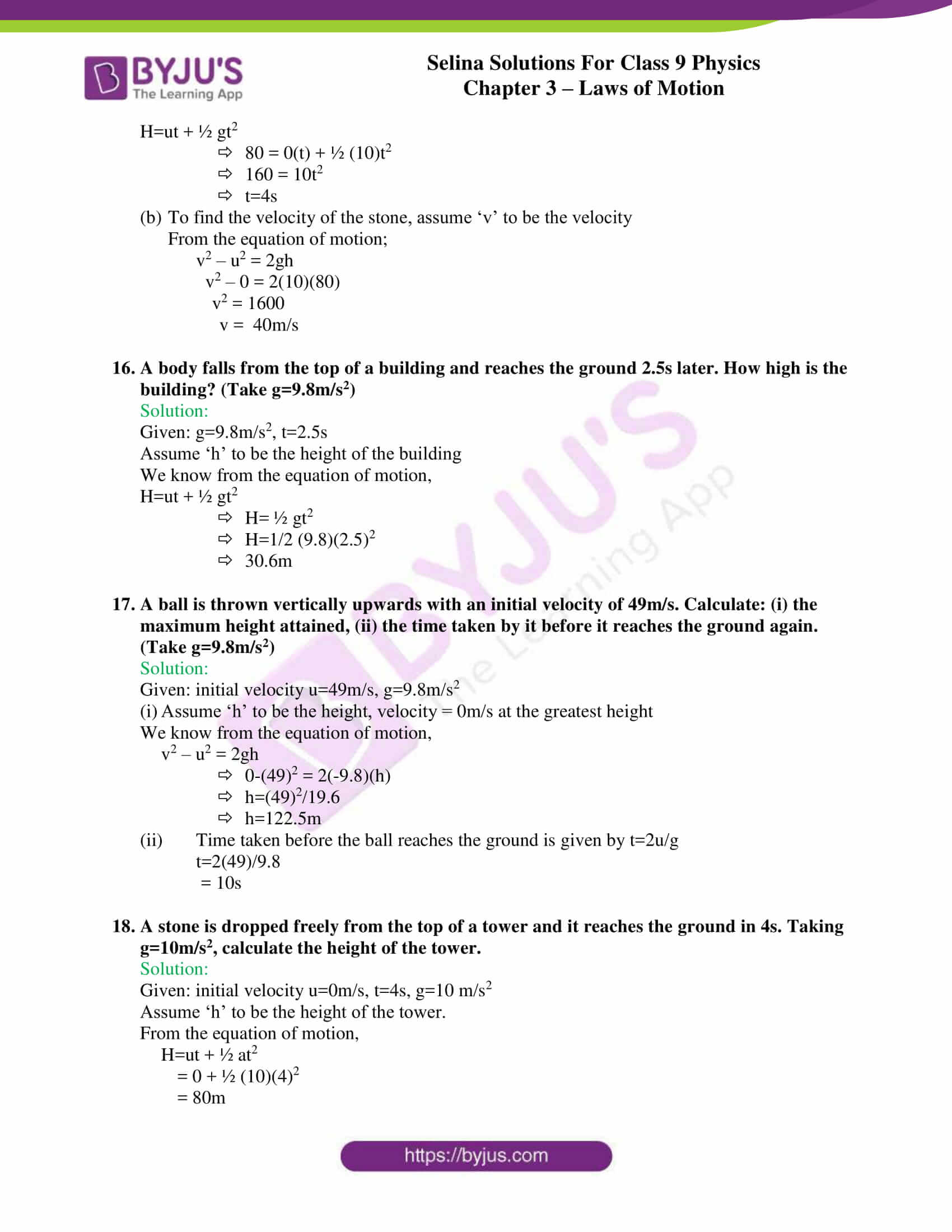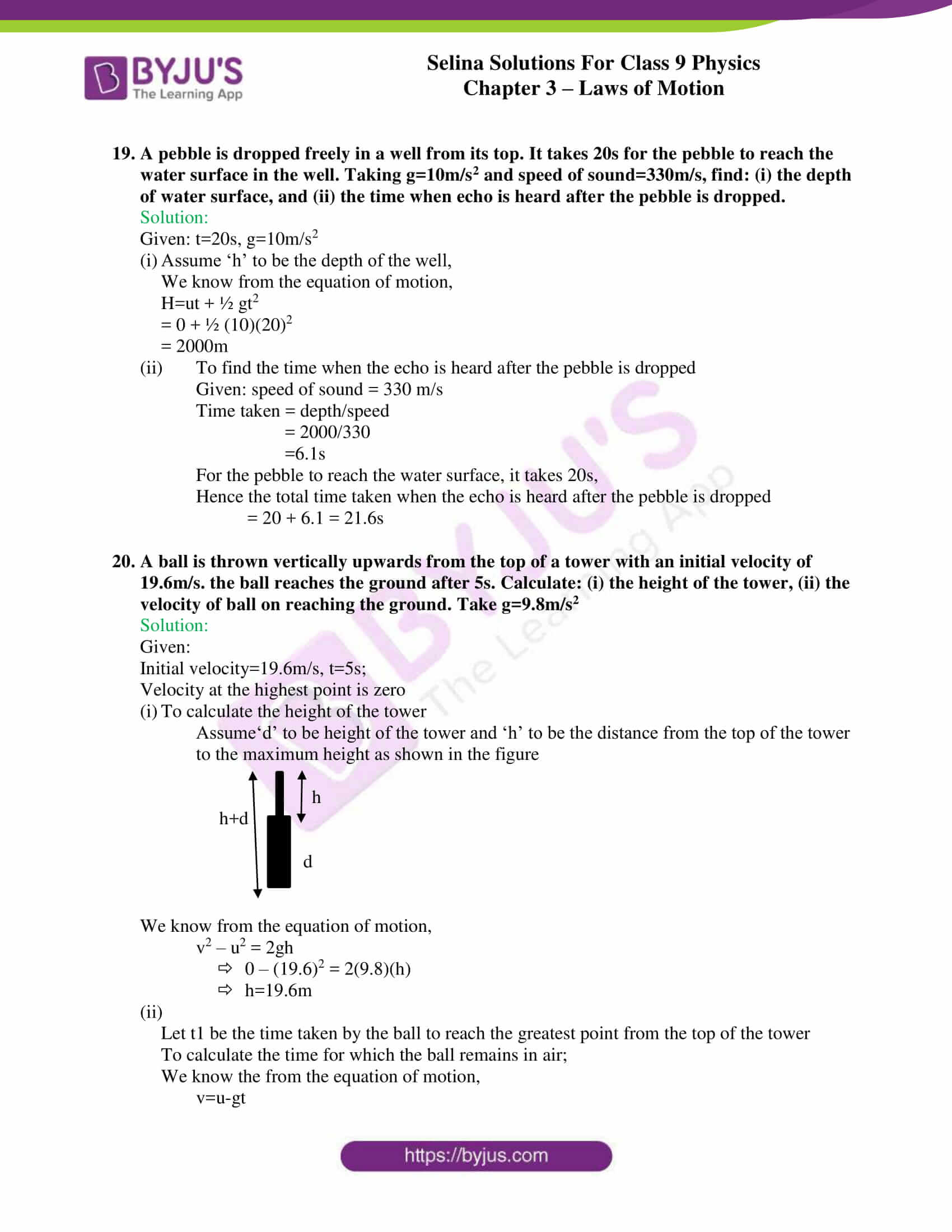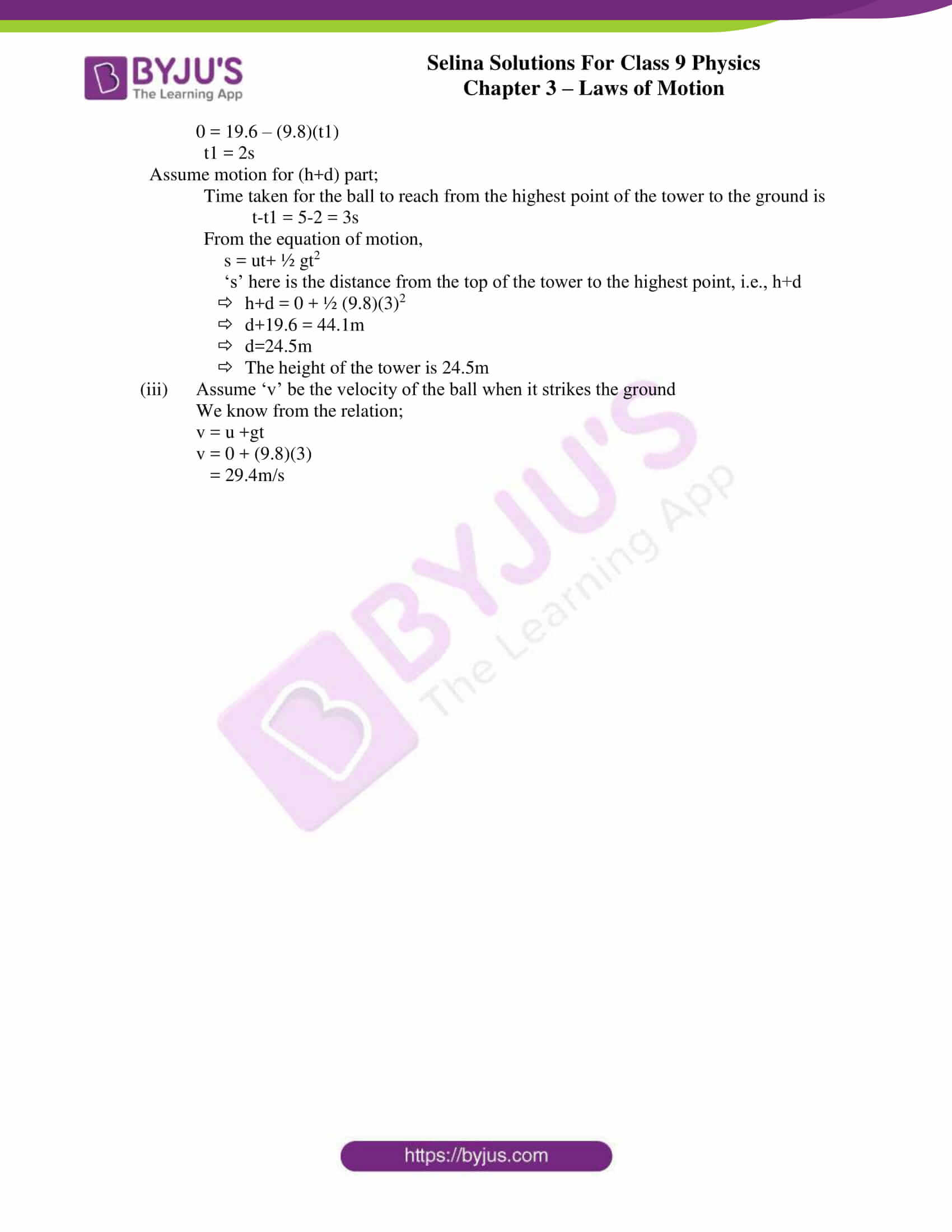## Selina Solutions for Class 9 Physics Chapter 3 – Laws of Motion

ICSE Class 9 Physics Chapter 3 – Laws of Motion is part of the syllabus for Class 9 students. The chapter has been included in order to facilitate the understanding of the different topics covered under the laws of motion, so as to cultivate a sense of practicality which is aided with a sufficient number of diagrams and graphs.

The chapter provides a brief description of various scientific ideologies in a simpler language without compromising on the information that is to be conveyed as per the standards. The chapter covers important concepts revolving around the laws of motion, the importance of different physical quantities and their role in understanding concepts pertaining to the laws. The central emphasis of the chapter lies on the concept of forces and how forces act on objects. Newton proposed three laws of motion, which have been clearly discussed and explained along with numericals for students to get a practical know-how of the concepts.

List of subtopics covered in Chapter 3 – Laws Of Motion:

 Number Subtopic 3.1 Effect of Force 3.2 Contact Forces 3.3 Non-Contact Forces 3.4 Newton’s First law of motion 3.5 Mass and Inertia 3.6 Kinds of inertia and its examples as illustration of first law 3.7 Linear Momentum 3.8 Change in momentum 3.9 Rate of change of momentum 3.10 Newton’s Second law of motion 3.11 C.G.S and S.I. units of force 3.12 Newton’s second law of motion in terms of rate of change of momentum 3.13 Newton’s third law of motion 3.14 Universal law of gravitation 3.15 Force due to gravity 3.16 Acceleration due to gravity 3.17 Free fall 3.18 Mass and weight 3.19 Gravitational units of force

List of Exercise

 Name of the exercise Number of questions Page number Exercise-3A 10 59 Exercise-3B 24 63 Exercise-3C 36 69 Exercise-3D 18 73 Exercise-3E 47 79

## Selina Solutions for Class 9 Physics Chapter 3 – Laws of Motion

Students through this chapter learn about the 3 fundamental laws of motion proposed by Newton. The chapter starts with a briefing about the two forces namely – contact and non-contact forces, discussing the various types of forces under each along with suitable examples.

It then advances towards the First law of motion wherein the topic is discussed qualitatively elucidated through the concepts of mass and inertia. Students are then provided with information on the linear momentum and the concept of change of momentum, soon after which Newton’s second law of motion along with the derivation is discussed.

Lastly, Newton’s third law of motion illustrates the significance of action and reaction and how the principle is applied in various fields today. Gravitation is another important concept that is discussed along with derivation and its relation to concepts, such as force due to gravity and acceleration due to gravity.

### Key Features of Selina Solutions for Class 9 Physics Chapter 3 – Laws Of Motion

• Selina solutions enable students to prepare for examination hassle-free.
• Solutions have been solved with the help of relevant examples
• Use of a well-marked graph wherever necessary
• Use of tables wherever necessary
• Solutions are framed in accordance with the information provided in the textbook
• Numericals are solved with complete explanations

## Frequently Asked Questions on Laws of Motion

### What are Contact forces?

These are the forces that act upon bodies when they are in physical contact, and are known as contact forces. Example-Force exerted on two bodies while the process of collision, frictional force.

### Is Frictional force a contact force?

Yes, Frictional force is a contact force. These are the forces that act upon bodies when they are in physical contact with each other.

### What are Non-Contact forces?

These are the forces that are experienced by the bodies without any physical contact, and are known as non-contact force. Example – Electrostatic force and gravitational force.

### Name the physical quantity which cause motion in a body?

The physical quantity that causes motion in a body is force.

### Is force needed to keep a moving body in motion?

No, force is not required to be applied to a moving body unless the direction or the speed is required to be changed.

### What is Galileo’s law of inertia?

Galileo’s law of inertia is as stated below:

“An object, if once set in motion, moves with uniform velocity if no force acts on it.”

### State Newton’s first law of motion?

Newton’s first law states that – if a body is in a state of rest, it will remain in the state of rest and if it is in the state of motion, it will remain moving in the same direction with the same speed unless an external force is applied on it.

### What is meant by the term inertia?

The property of an object by virtue of which it tends to retain its state of rest or of uniform is called inertia.

### Name the two kinds of inertia.

The two kinds of inertia are:

• Inertia of rest
• Inertia of motion